• LFMCW雷达测距测速，代码中有详细注释。汽车安装线性调频连续波雷达测距测速
• 线性调频脉冲压缩雷达仿真及matlab代码 1.线性调频脉冲雷达的工作原理 2.线性调频信号（LFM信号）时域频域仿真 3.脉冲压缩技术（时域压缩，频域压缩，多种方法） 4.雷达测距，脉冲压缩技术 5.雷达测速，脉冲压缩技术...
**阅读须知：**本文摘取自AIMZZY原创文章，并做改动，在此致谢
原文链接：https://blog.csdn.net/AIMZZY/article/details/106467080
线性调频脉冲压缩雷达仿真1.线性调频脉冲雷达的工作原理2.线性调频信号（LFM信号）2.1.LFM信号（时域分析）2.1.1.LFM信号模型2.1.2.LFM信号时域仿真2.2.LFM信号（频域分析）2.2.1.驻定相位原理（POSP）2.2.2.LFM信号频谱仿真3.脉冲压缩技术3.1.匹配滤波器3.1.1.匹配滤波器的推导3.1.2.匹配滤波器的理解3.2.LFM信号脉冲压缩的匹配滤波器实现3.2.1.时域匹配滤波3.2.2.频域匹配滤波3.2.2.1.方法一3.2.2.2.方法二3.2.2.3.方法三3.3.LFM脉冲压缩加窗处理3.3.1.性能指标3.3.2.各类窗的对比3.3.3.脉冲压缩加窗3.3.3.1.Hanning窗脉冲压缩加窗举例3.3.3.2.Blackman窗脉冲压缩加窗举例4.LFM脉冲压缩雷达测距4.1.测距雷达的任务要求：4.2.系统模型的构建4.3.关于距离分辨率4.4.最终效果呈现4.5.LFM脉冲压缩雷达测距matlab代码5.脉冲多普勒雷达同时实现测距测速5.1.任务介绍5.2.matlab代码5.2.测距测速结果展示5.2.1.情况（1）5.2.2.其他情况6.脉冲多普勒雷达距离速度三维像6.1.结合第五部分实现三维信息表示：6.2.matlab代码实现
1.线性调频脉冲雷达的工作原理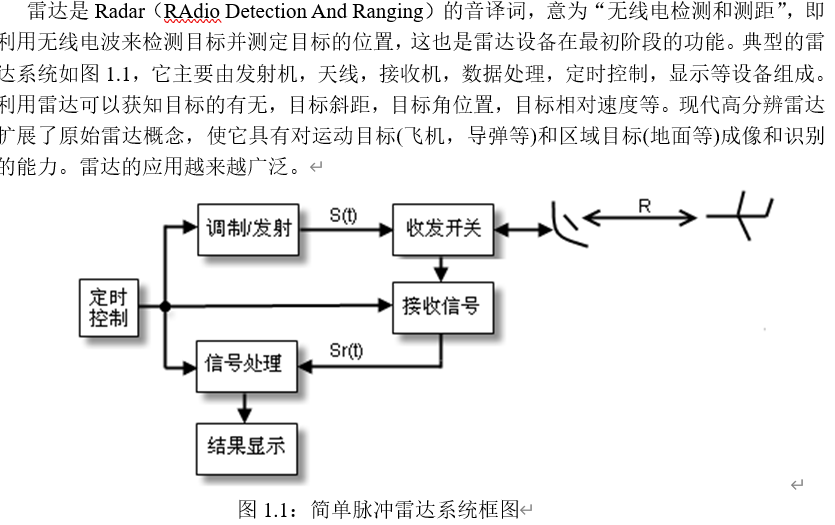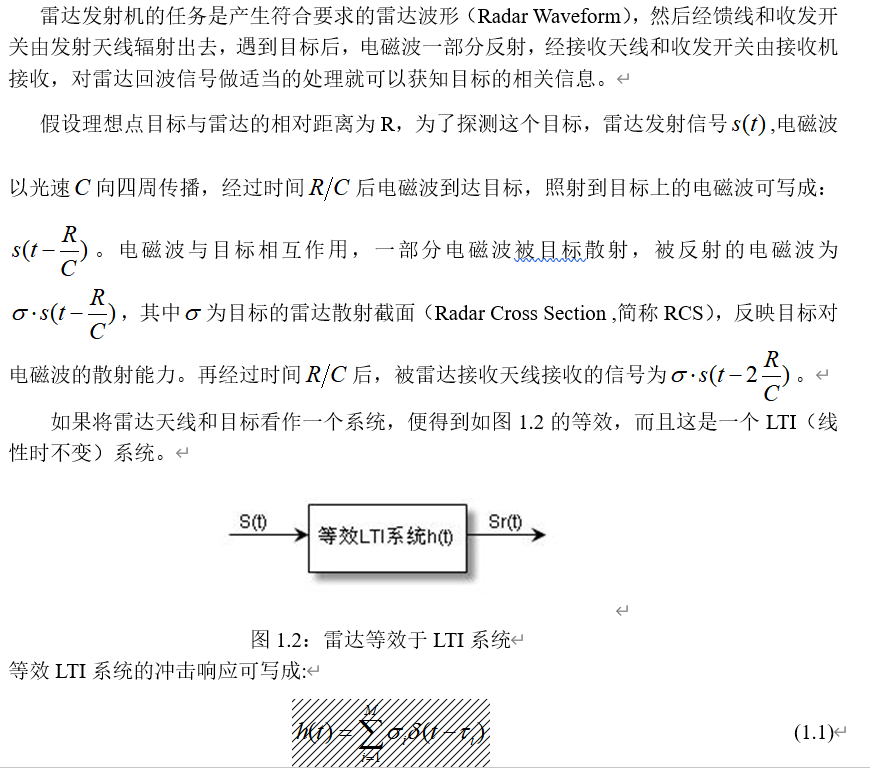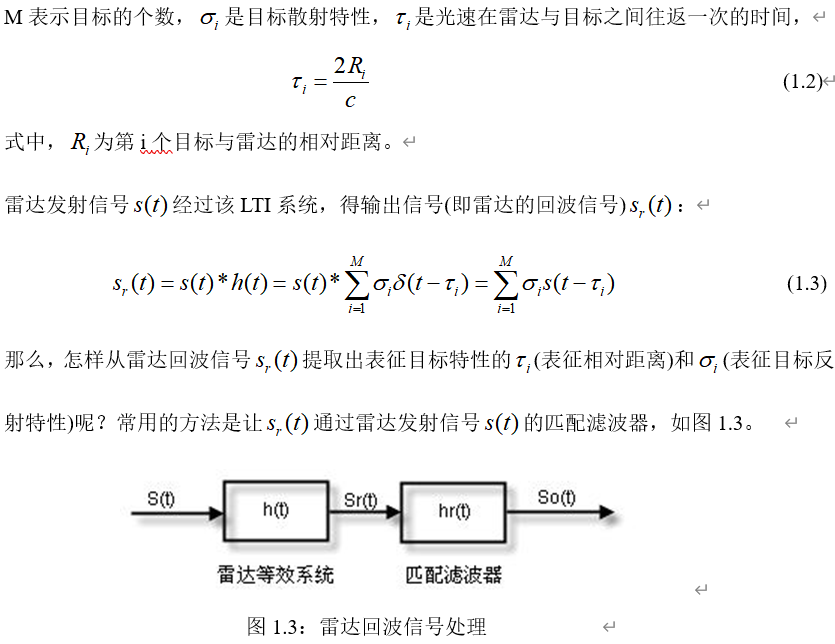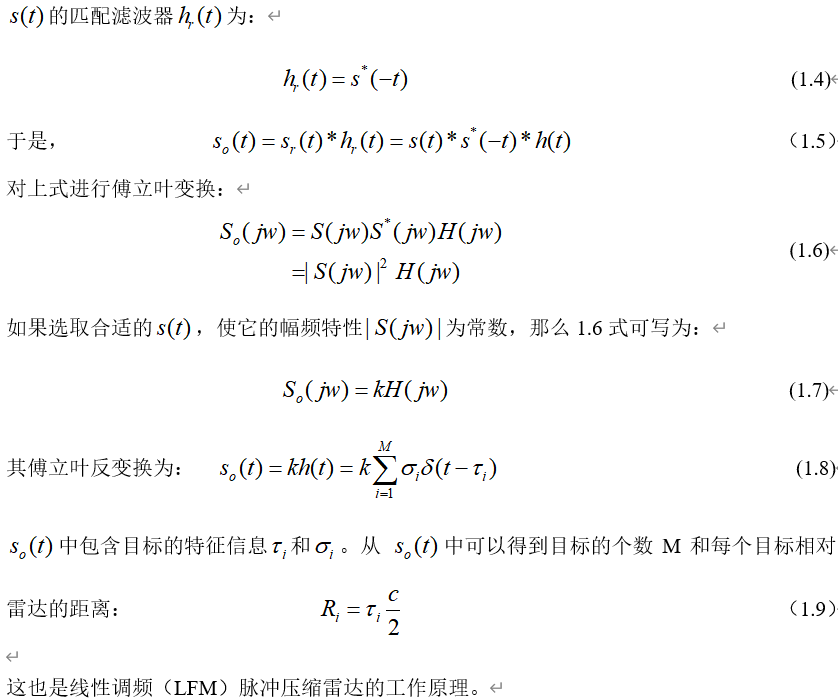2.线性调频信号（LFM信号）
2.1.LFM信号（时域分析）
2.1.1.LFM信号模型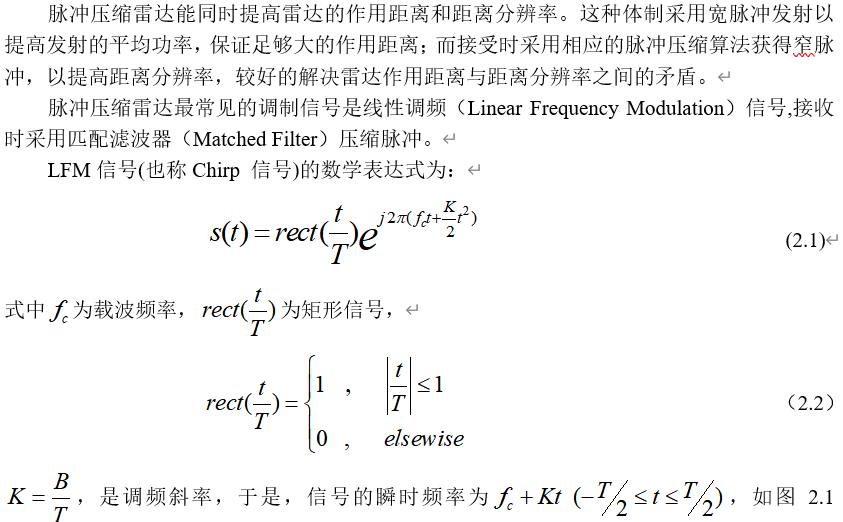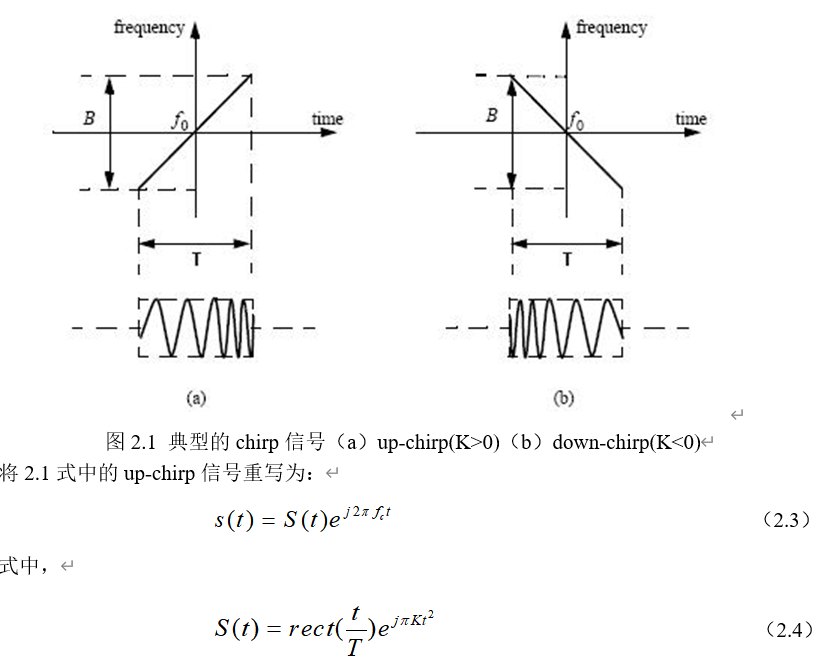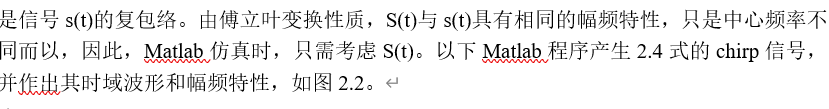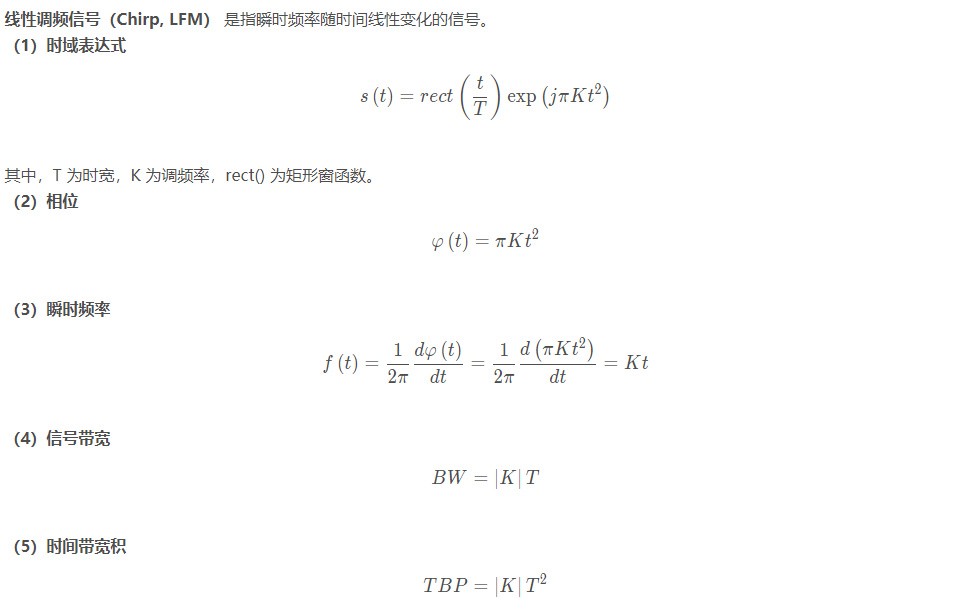2.1.2.LFM信号时域仿真%%线性调频信号
T=10e-6;                                  %p脉冲持续时间10us
B=30e6;                                   %线性调频信号的频带宽度30MHz
K=B/T;                                      %调频斜率
Fs=2*B;Ts=1/Fs;                      %采样频率和采样间隔
N=T/Ts;
t=linspace(-T/2,T/2,N);
St=exp(j*pi*K*t.^2);                    %线性调频信号
subplot(211)
plot(t*1e6,real(St));
xlabel('时间/us');
title('线性调频信号的实部');
grid on;axis tight;
subplot(212)
freq=linspace(-Fs/2,Fs/2,N);
plot(freq*1e-6,fftshift(abs(fft(St))));
xlabel('频率/MHz');
title('线性调频信号的幅频特性');
grid on;axis tight;

（1）信号参数
① 时宽 Tr = 1 us
② 带宽 Br = 100 MHz
③ 采样率 Fs = 4 * Br
（2）仿真结果

信号实部
信号虚部
信号频率
column4%% 线性调频与脉冲压缩
clear,clc,close all
set(0,'defaultfigurecolor','w')
%% Chirp信号参数设置
Tr = 1e-6;%时宽
Br = 200e6;%带宽
Fs = 4*Br;%采样率
%% Chirp信号参数导出
Kr = Br/Tr;%调频率
N =  round( Tr / (1/Fs) );%采样点数
t = linspace( -Tr/2 , Tr/2 , N);%在[-Tp/2,Tp/2]选取采样点
%% Chirp信号生成
st = ( abs(t) < Tr/2 ) .* exp( 1j * pi * Kr * t.^2 );
f_chirp= Kr * t; %信号频率
phase_chirp = pi * Kr * t.^2;%信号相位
% 时域
figure,plot( t*1e6, real(st) ),xlabel('t /us'),ylabel('幅度'),title('Chirp信号实部');
figure,plot( t*1e6, imag(st) ),xlabel('t /us'),ylabel('幅度'),title('Chirp信号虚部');
figure,plot( t*1e6, f_chirp/1e6 ),xlabel('t /us'),ylabel('频率 /MHz'),title('Chirp信号频率');
figure,plot( t*1e6, phase_chirp ),xlabel('t /us'),ylabel('相位 /rad'),title('Chirp信号相位');


2.2.LFM信号（频域分析）
2.2.1.驻定相位原理（POSP）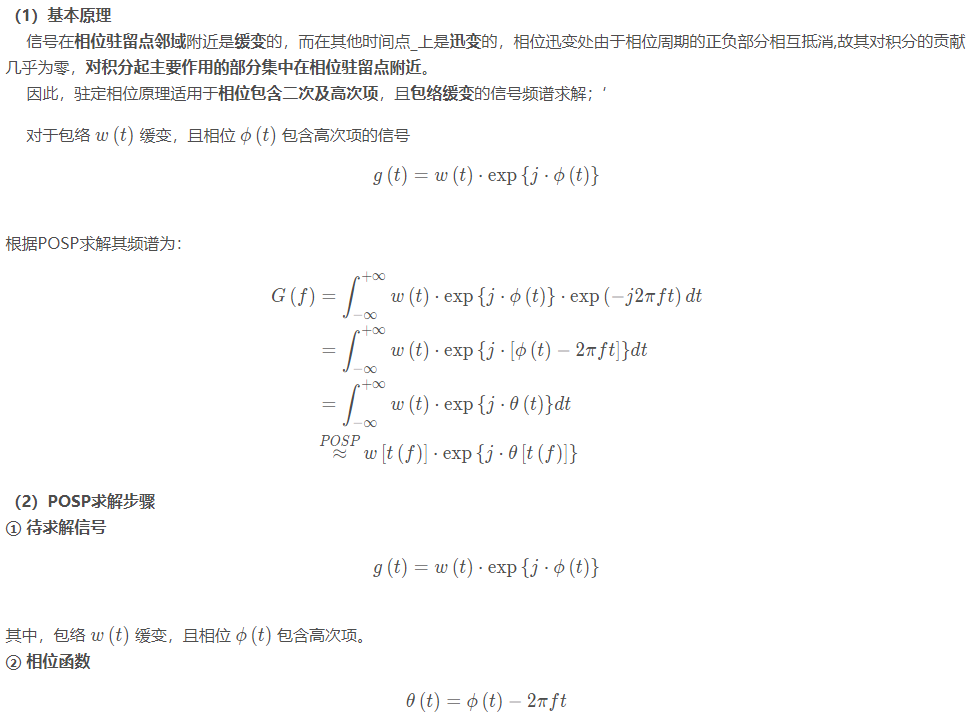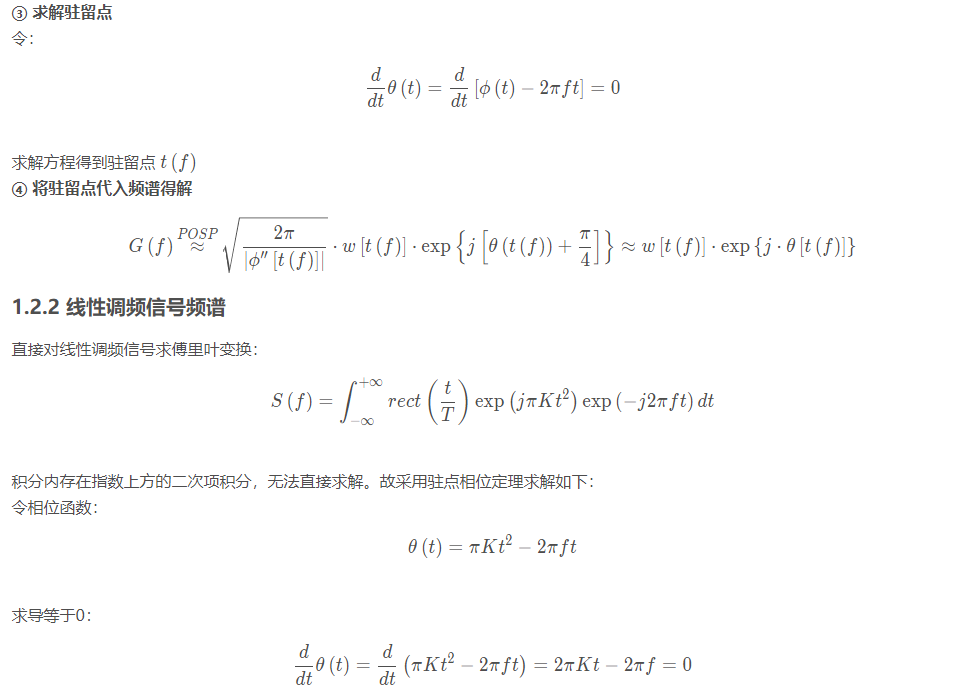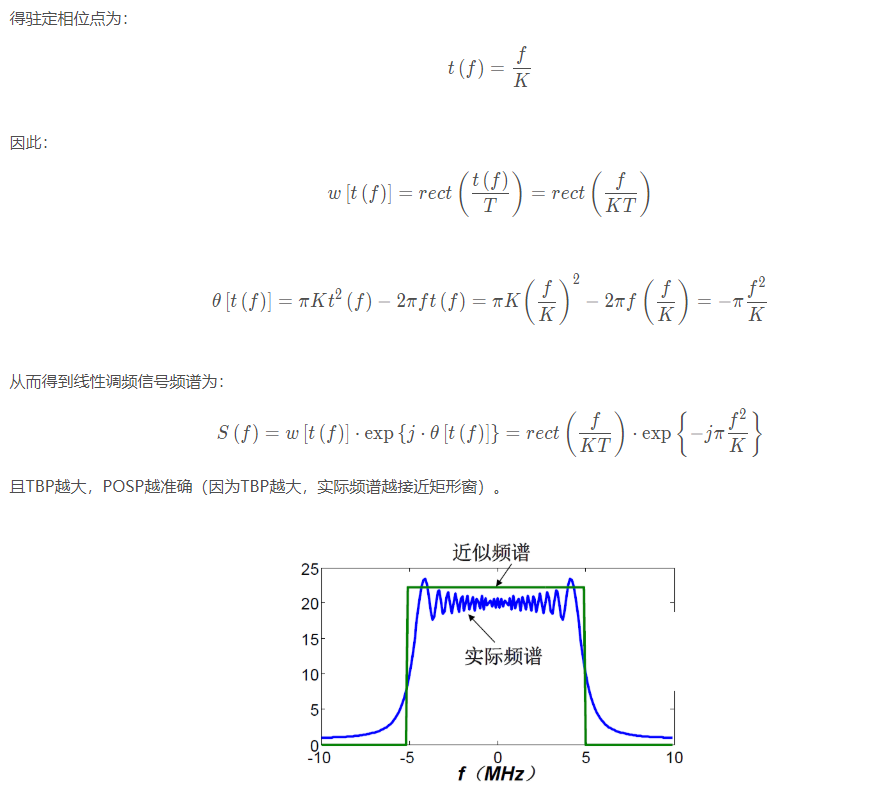2.2.2.LFM信号频谱仿真

频谱幅度谱
频谱相位谱%% 线性调频与脉冲压缩
clear,clc,close all
set(0,'defaultfigurecolor','w')
%% Chirp信号参数设置
Tr = 1e-6;%时宽
Br = 200e6;%带宽
Fs = 4*Br;%采样率
%% Chirp信号参数导出
Kr = Br/Tr;%调频率
N =  round( Tr / (1/Fs) );%采样点数
t = linspace( -Tr/2 , Tr/2 , N);%在[-Tp/2,Tp/2]选取采样点
%% Chirp信号生成
st = ( abs(t) < Tr/2 ) .* exp( 1j * pi * Kr * t.^2 );
f_chirp= Kr * t; %信号频率
phase_chirp = pi * Kr * t.^2;%信号相位
%% 频谱
freq = linspace(-Fs/2,Fs/2,N);%频域采样
Sf = fftshift( fft(st) );
% 频域
figure,plot( freq/1e6,abs(Sf) ),xlabel('f /MHz'),ylabel('幅度谱'),title('Chirp信号 幅度谱');
figure,plot( freq/1e6,-pi*freq.^2/Kr ),xlabel('f /MHz'),ylabel('相位谱'),title('Chirp信号 相位谱');

3.脉冲压缩技术
3.1.匹配滤波器
3.1.1.匹配滤波器的推导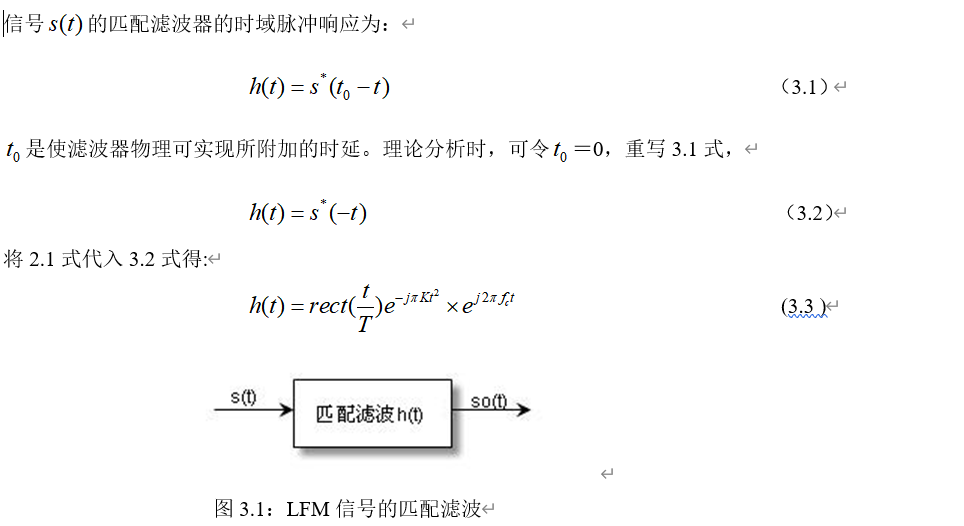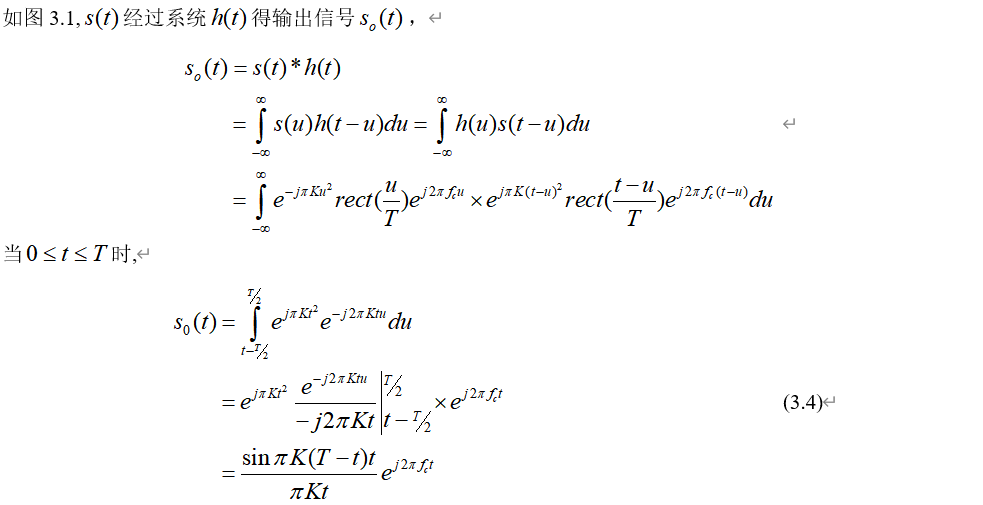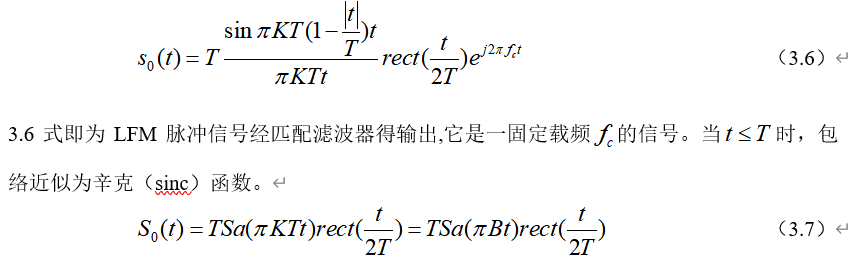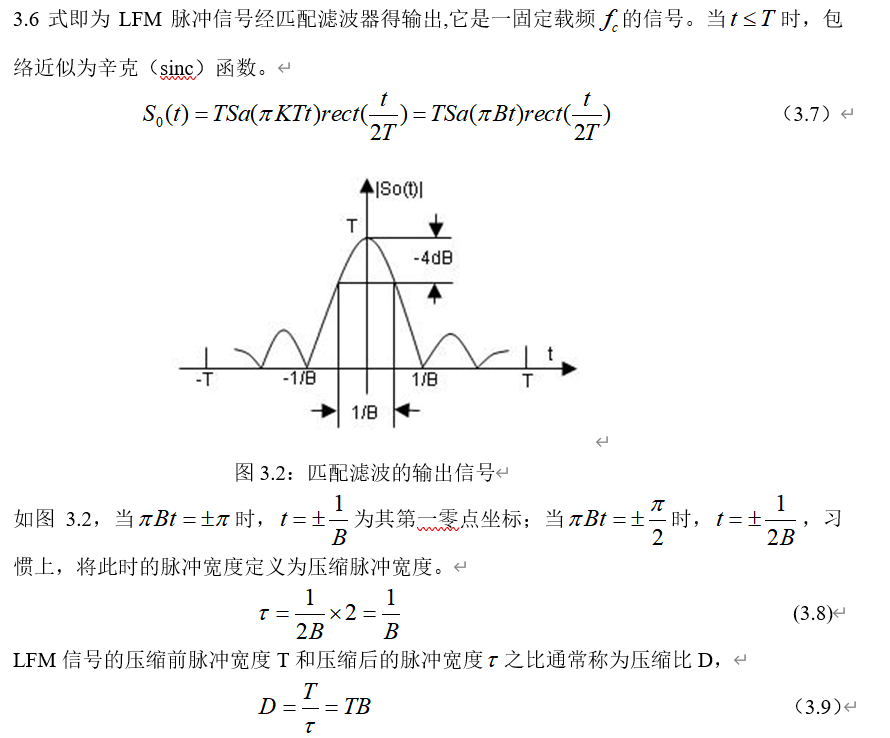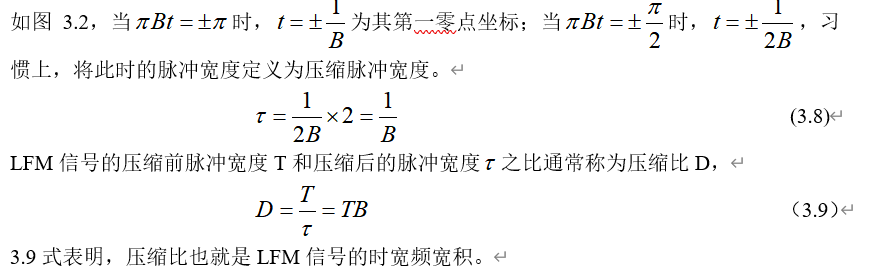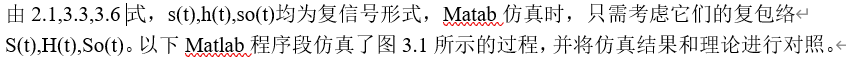3.1.2.匹配滤波器的理解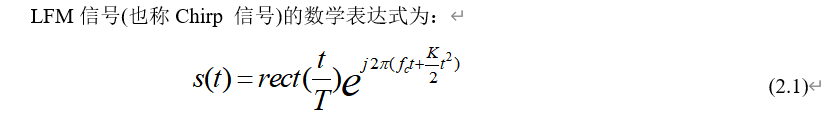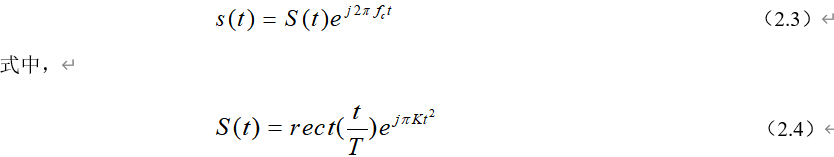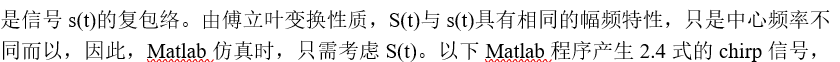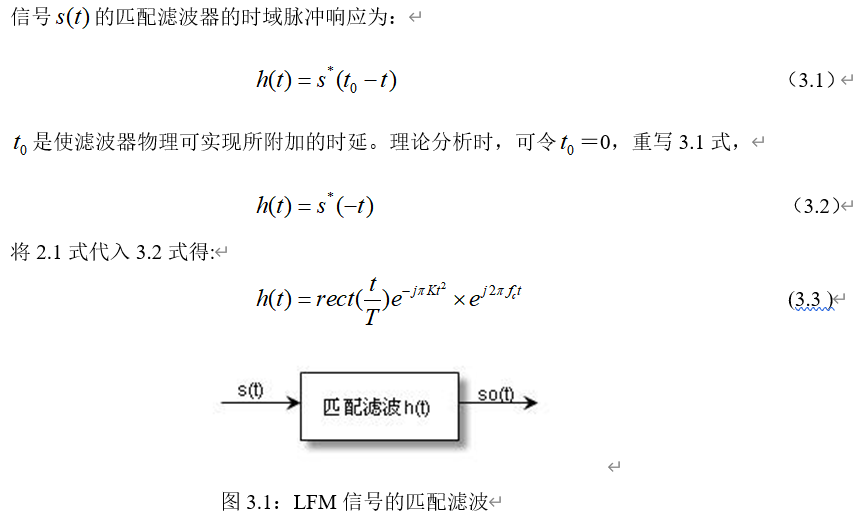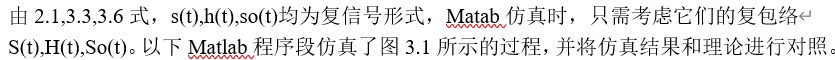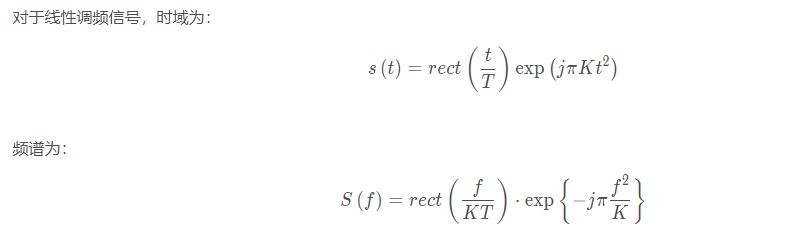3.2.LFM信号脉冲压缩的匹配滤波器实现
3.2.1.时域匹配滤波%% 线性调频与脉冲压缩
clear,clc,close all
set(0,'defaultfigurecolor','w')
%% Chirp信号参数设置
Tr = 1e-6;%时宽
Br = 200e6;%带宽
Fs = 4*Br;%采样率
%% Chirp信号参数导出
Kr = Br/Tr;%调频率
N =  round( Tr / (1/Fs) );%采样点数
t = linspace( -Tr/2 , Tr/2 , N);%在[-Tp/2,Tp/2]选取采样点
%% Chirp信号生成
st = ( abs(t) < Tr/2 ) .* exp( 1j * pi * Kr * t.^2 );
f_chirp= Kr * t; %信号频率
phase_chirp = pi * Kr * t.^2;%信号相位
%% 频谱
freq = linspace(-Fs/2,Fs/2,N);%频域采样
Sf = fftshift( fft(st) );
%% 时域匹配滤波
ht = conj( fliplr(st) ); %时域匹配滤波为发射信号时间反褶再取共轭
s1 = conv(st,ht); %线性调频信号经过匹配滤波器后的输出(时域卷积)
N1 = N+N-1 ;%线性卷积后信号长度变为 N1+N2-1
t1 = linspace( -Tr/2 , Tr/2 , N1);
% 时域匹配滤波
figure,plot( t1*1e6 , abs(s1) ),xlabel('t /us'),ylabel('幅度谱'),title('时间反褶取共轭，时域卷积');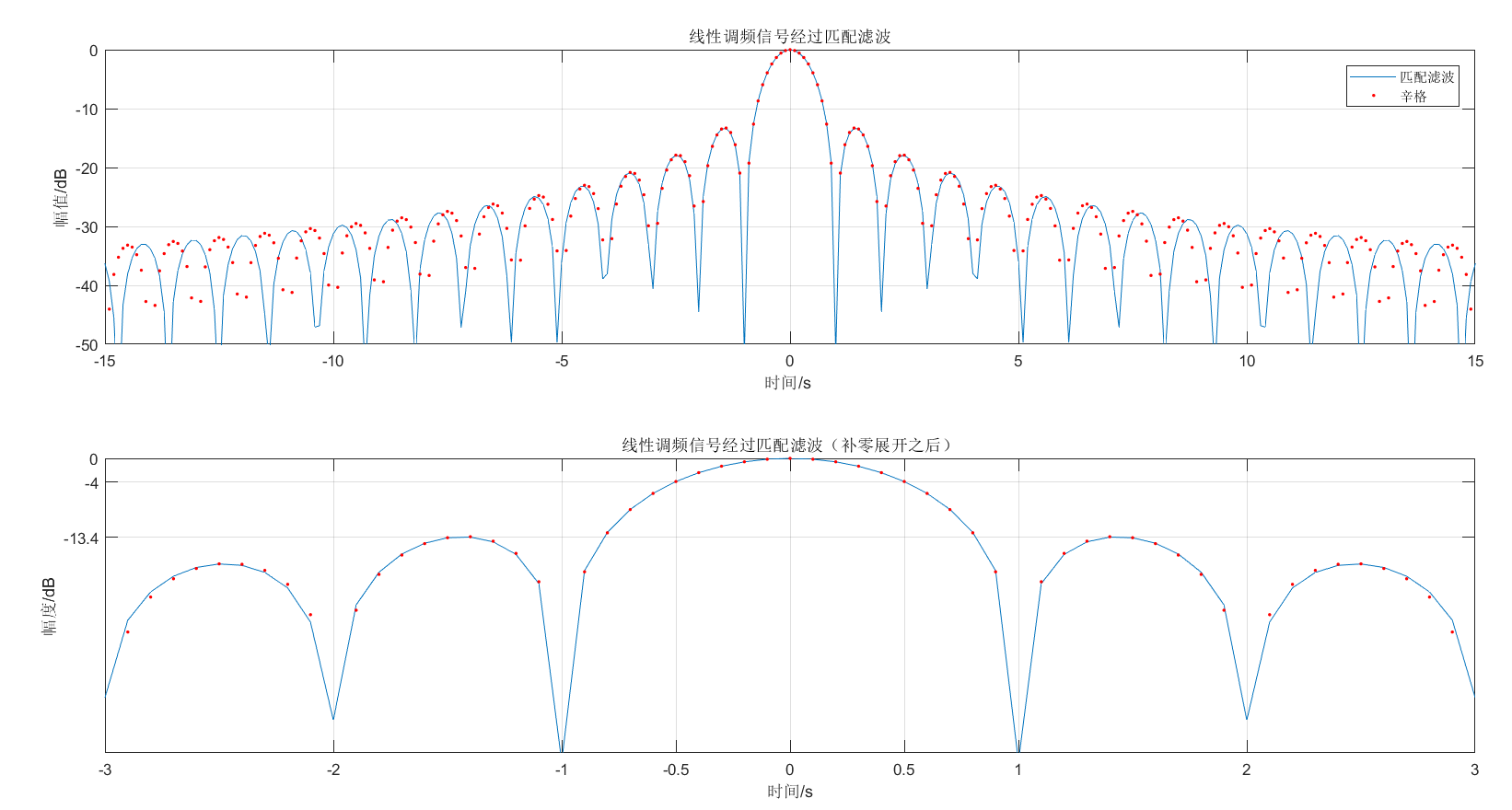%%线性调频信号经过匹配滤波
T=10e-6;                                   %脉冲持续时间10us
B=30e6;                                    %线性调频信号的带宽30MHz
K=B/T;                                     %调频斜率
Fs=10*B;Ts=1/Fs;                     %采样频率和采样间隔
N=T/Ts;
t=linspace(-T/2,T/2,N);
St=exp(j*pi*K*t.^2);                     %线性调频信号
Ht=exp(-j*pi*K*t.^2);                    %匹配滤波
Sot=conv(St,Ht);                         %线性调频信号经过匹配滤波
subplot(211)
L=2*N-1;
t1=linspace(-T,T,L);
Z=abs(Sot);Z=Z/max(Z);             %归一化
Z=20*log10(Z+1e-6);
Z1=abs(sinc(B.*t1));                   %辛格函数
Z1=20*log10(Z1+1e-6);
t1=t1*B;
plot(t1,Z,t1,Z1,'r.');
axis([-15,15,-50,inf]);grid on;
legend('匹配滤波','辛格');
xlabel('时间/s ');
ylabel('幅值/dB');
title('线性调频信号经过匹配滤波');
subplot(212)                              %展开
N0=3*Fs/B;
t2=-N0*Ts:Ts:N0*Ts;
t2=B*t2;
plot(t2,Z(N-N0:N+N0),t2,Z1(N-N0:N+N0),'r.');
axis([-inf,inf,-50,inf]);grid on;
set(gca,'Ytick',[-13.4,-4,0],'Xtick',[-3,-2,-1,-0.5,0,0.5,1,2,3]);
xlabel('时间/s');
ylabel('幅度/dB');
title('线性调频信号经过匹配滤波（补零展开之后）');

3.2.2.频域匹配滤波
3.2.2.1.方法一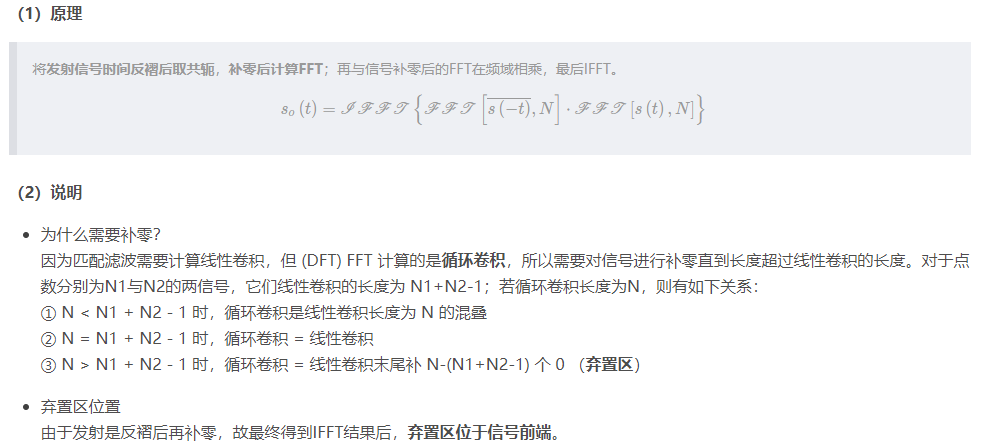%% 频域匹配滤波1 (复制发射脉冲进行时间反褶并取共轭，计算补零DFT)
N2 = 2*N; %循环卷积长度 （N2应当>=N+N-1，其中弃置区位于长度大于N+N-1的部分）
t2 = linspace( -Tr/2 , Tr/2 , N2);
Hf2 = fft(ht,N2); %频域匹配滤波器
Sf2 = fft(st,N2);%频域信号
S2 = Sf2 .* Hf2;%频域乘积
s2 = ifft(S2);
% 频域匹配滤波1
figure,plot( t2*1e6 , abs(s2) ),xlabel('t /us'),ylabel('幅度谱'),title('时间反褶取共轭，补零FFT，频域乘积，IFFT');

3.2.2.2.方法二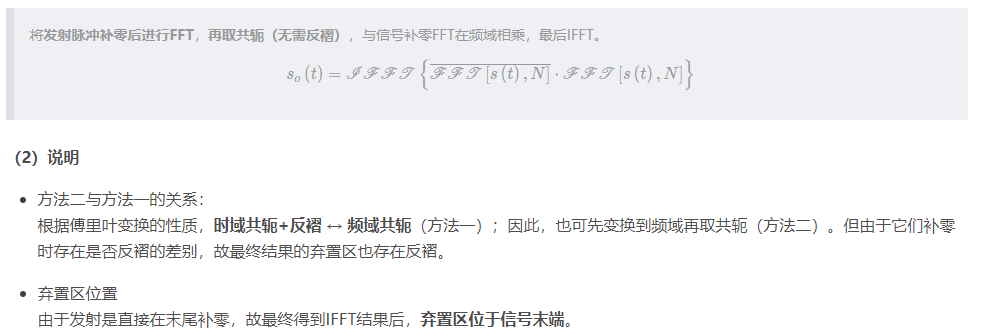%% 频域匹配滤波2（复制脉冲补零后进行DFT，对结果取复共轭（无时间反褶））
N3 = 2*N; %循环卷积长度
t3 = linspace( -Tr/2 , Tr/2 , N3);
Hf3 = conj( fft(st,N3) );% 复制脉冲补零后进行DFT，对结果取复共轭
Sf3 = fft(st,N3);
S3 = Sf3 .* Hf3;%频域乘积
s3 = fftshift(ifft(S3));
% 频域匹配滤波2
figure,plot( t3*1e6 , abs(s3) ),xlabel('t /us'),ylabel('幅度谱'),title('复制脉冲补零后FFT，取共轭，频域乘积，IFFT');

3.2.2.3.方法三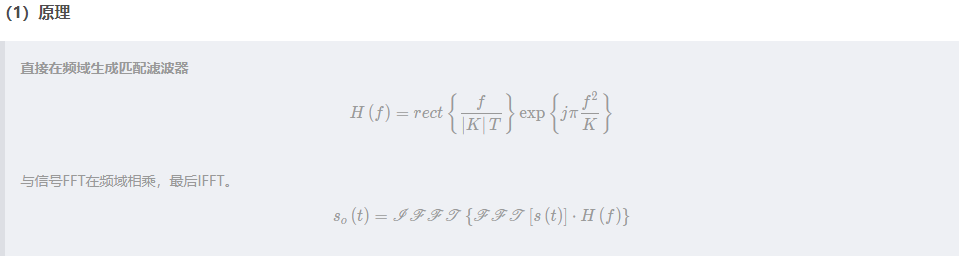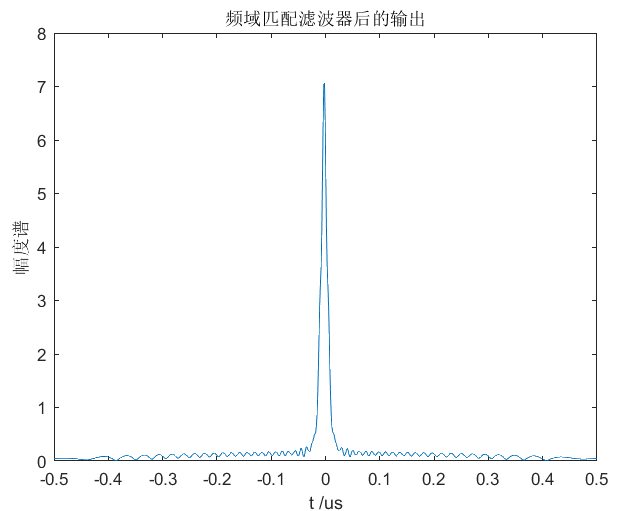3.3.LFM脉冲压缩加窗处理
3.3.1.性能指标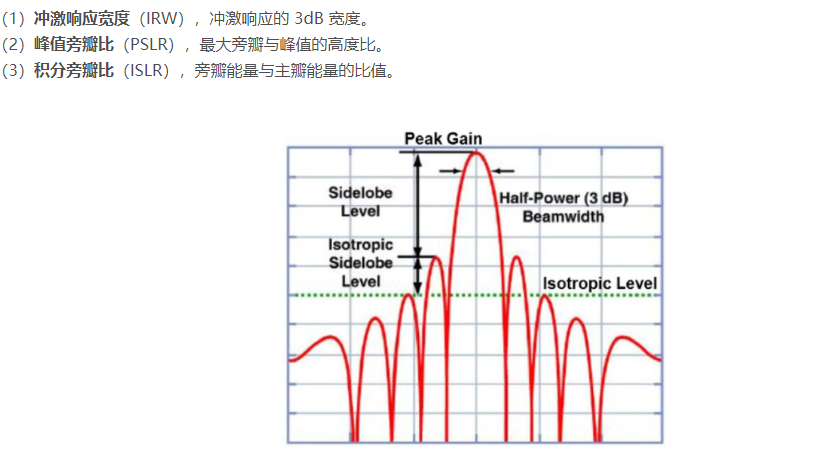3.3.2.各类窗的对比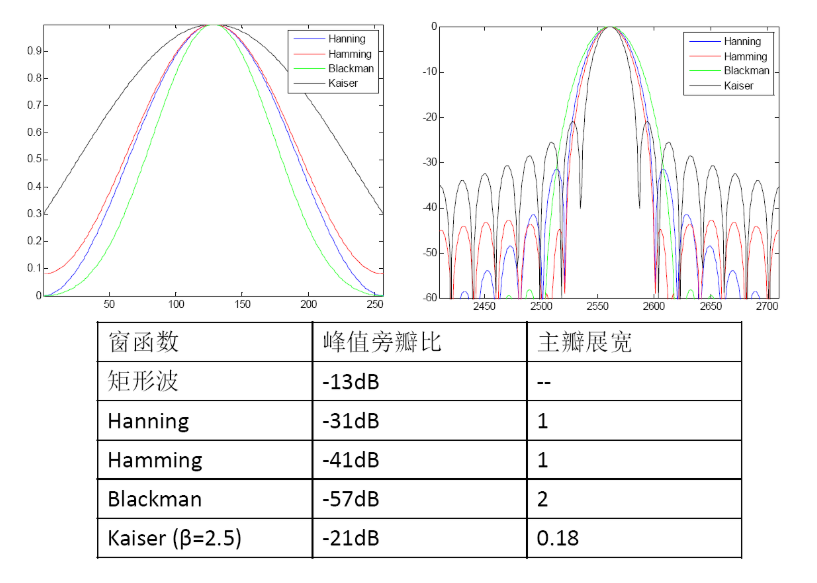3.3.3.脉冲压缩加窗
3.3.3.1.Hanning窗脉冲压缩加窗举例

没有加汉宁窗 脉冲压缩
加汉宁窗脉冲压缩
两者进行对此%%%%%%%  利用频域处理方法进行脉冲压缩  %%%%%%%
clear all
clc
clf
eps = 1e-10;
B=100e-6;       %信号带宽
Fm=1e6;           %调频
k=Fm/B;          %调频斜率
Ts=1/(5*Fm);        %采样周期
Ns=fix(B/Ts);        %采样点数
Nf=1024;               % fft点数
t=0:Ts:B-Ts;
y=exp(j*pi*k*t.^2);   %脉冲压缩前的线形调频信号
yfft = fft(y,Nf) ;
h=zeros(1,Ns);
%% %%%%%%%%%%%%%%%%%%%%%%%%%Hamming窗%%%%%%%%%%%%%%%%%%%%%%%%%%%
for i=1:Ns
h(i)=conj(y(Ns-i+1));
end
hfft= fft(h,Nf);     % 匹配滤波器的频域响应
lfm =abs(ifft(yfft .*hfft)); %脉冲压缩
maxval = max (lfm);
lfm = eps + lfm ./ maxval;    % 利用最大值归一化
lfm_db=20*log10(lfm);   %取对数
%%%%%%%%%%%%%% 加窗处理 %%%%%%%
win = hamming(Ns)';
h_w=h.*win;       % 加窗
hfft_w=fft(h_w,Nf);     % 加窗的匹配滤波器的频域响应
lfm_w = abs(ifft(yfft .*hfft_w)); %脉冲压缩
maxval1 = max(lfm_w);
val=lfm_w ;
lfm_w = eps + lfm_w ./ maxval;    % 利用lfm的最大值归一化
lfm_w1 = eps + val./ maxval1;    % 利用lfm_w的最大值归一化
lfm_w_db=20*log10(lfm_w);   %取对数
lfm_w1_db=20*log10(lfm_w1);   %取对数
%%%%%%%%%%%%%%%%
tt =0:Ts:2*B-Ts;
figure(1)
plot (tt,lfm_db(1:2*Ns),'b')
axis([.2*B 1.8*B -60 0] )
xlabel ('t - seconds ');
ylabel(' db')
title('没有加Hamming窗的脉冲压缩输出')
grid on
figure(2)
plot (tt,lfm_w1_db(1:2*Ns),'r')
axis([.2*B 1.8*B -60 0] )
xlabel ('t - seconds ');
ylabel(' db')
title('加Hamming窗的脉冲压缩输出')
grid on
figure(3)
plot (tt,lfm_db(1:2*Ns),'b',tt,lfm_w_db(1:2*Ns),'r')
axis([.7*B  1.3*B -60 0] )
xlabel ('t - seconds ');
ylabel(' db')
legend('未加Hamming窗','加Hamming窗');
title('脉冲压缩输出对比')
grid on

3.3.3.2.Blackman窗脉冲压缩加窗举例

不加窗脉冲压缩
加布莱克曼
两者对比%% %%%%%%%%%%%%%%%%%%%%%%%%%Blackman窗%%%%%%%%%%%%%%%%%%%%%%%%%%%
clc
clf
eps = 1e-10;
B=100e-6;       %信号带宽
Fm=1e6;           %调频
k=Fm/B;          %调频斜率
Ts=1/(5*Fm);        %采样周期
Ns=fix(B/Ts);        %采样点数
Nf=1024;               % fft点数
t=0:Ts:B-Ts;
y=exp(j*pi*k*t.^2);   %脉冲压缩前的线形调频信号
yfft = fft(y,Nf) ;
h=zeros(1,Ns);
for i=1:Ns
h(i)=conj(y(Ns-i+1));
end
hfft= fft(h,Nf);     % 匹配滤波器的频域响应
lfm =abs(ifft(yfft .*hfft)); %脉冲压缩
maxval = max (lfm);
lfm = eps + lfm ./ maxval;    % 利用最大值归一化
lfm_db=20*log10(lfm);   %取对数
%%%%%%%%%%%%%% 加窗处理 %%%%%%%
win = blackman(Ns)';
h_w=h.*win;       % 加窗
hfft_w=fft(h_w,Nf);     % 加窗的匹配滤波器的频域响应
lfm_w = abs(ifft(yfft .*hfft_w)); %脉冲压缩
maxval1 = max(lfm_w);
val=lfm_w ;
lfm_w = eps + lfm_w ./ maxval;    % 利用lfm的最大值归一化
lfm_w1 = eps + val./ maxval1;    % 利用lfm_w的最大值归一化
lfm_w_db=20*log10(lfm_w);   %取对数
lfm_w1_db=20*log10(lfm_w1);   %取对数
%%%%%%%%%%%%%%%%
tt =0:Ts:2*B-Ts;
figure(1)
plot (tt,lfm_db(1:2*Ns),'b')
axis([.2*B 1.8*B -60 0] )
xlabel ('t - seconds ');
ylabel(' db')
title('没有加Blackman窗的脉冲压缩输出')
grid on
figure(2)
plot (tt,lfm_w1_db(1:2*Ns),'r')
axis([.2*B 1.8*B -60 0] )
xlabel ('t - seconds ');
ylabel(' db')
title('加Blackman窗的脉冲压缩输出')
grid on
figure(3)
plot (tt,lfm_db(1:2*Ns),'b',tt,lfm_w_db(1:2*Ns),'r')
axis([.7*B  1.3*B -60 0] )
xlabel ('t - seconds ');
ylabel(' db')
legend('未加Blackman窗','加Blackman窗');
title('脉冲压缩输出对比')
grid on

4.LFM脉冲压缩雷达测距
4.1.测距雷达的任务要求：4.2.系统模型的构建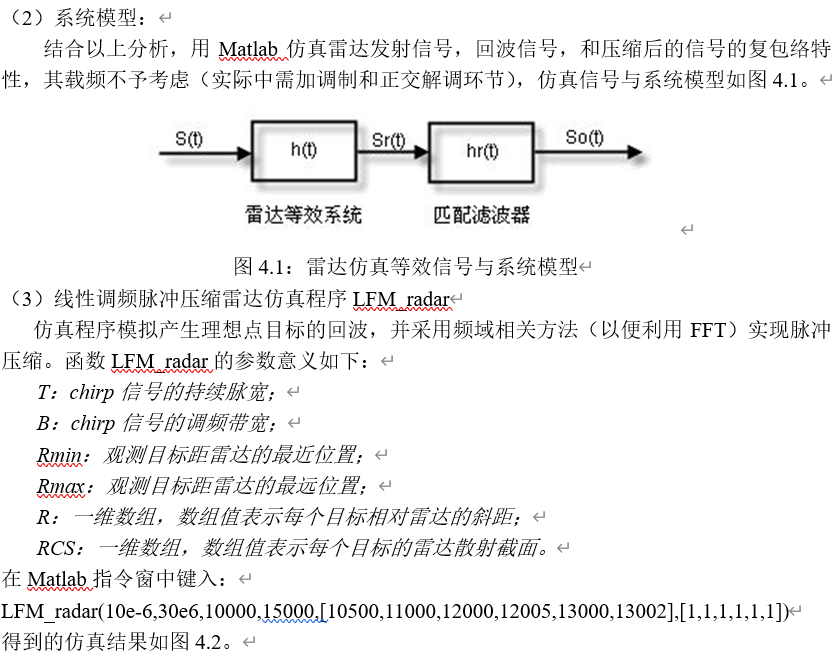4.3.关于距离分辨率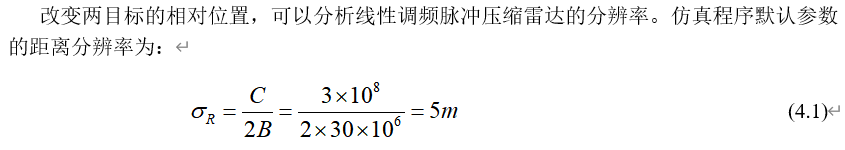两者距离
分辨情况

2m5m7m8m9m10m4.4.最终效果呈现
之后我们设置6个目标，目标的雷达截面积都为1，目标假设都为静止，我们根据雷达的相关参数设置回波窗的长度，同时根据雷达的测距范围设置6个目标物的距离，之后进行仿真，分别求出雷达回波以及经过脉冲匹配之后的结果，看是否能对目标实现测距，结果如下图所示：4.5.LFM脉冲压缩雷达测距matlab代码
%% LFM 脉冲多普勒雷达
%==================================================================
if nargin==0
T=10e-6;                                          %脉冲宽度10us
Tr=100e-6;                                          %脉冲重复周期
B=30e6;                                           %频带宽度30MHz
Rmin=10000;Rmax=15000;              %测距范围
%     R=[13000,13500];%目标点的位置，每一个目标相对于雷达的斜距
%     RCS=[1,1];                           %雷达截面积，一维数组
RCS=[1,1,1,1,1,1];
R=[10500,11000,12000,12008,13000,13002];  %目标点的位置，每一个目标相对于雷达的斜距
RCS=[1 1 1 1 1 1];   %雷达截面积，一维数组
end
%====================================
%%
C=3e8;                                            %光速
K=B/T;                                             %调频斜率
Rwid=Rmax-Rmin;                           %最大测距长度
Twid=2*Rwid/C;                               %回波窗的长度
Fs=5*B;Ts=1/Fs;                             %采样频率与采样时间
Nwid=ceil(Twid/Ts);                         %采样窗内的采样点数
%==================================================================
%%产生回拨
t=linspace(2*Rmin/C,2*Rmax/C,Nwid); %回波窗
%open window when t=2*Rmin/C
%close window when t=2*Rmax/C
M=length(R);                                        %目标的个数
td=ones(M,1)*t-2*R'/C*ones(1,Nwid);
Srt=RCS*(exp(j*pi*K*td.^2).*(abs(td)<T/2));%从点目标来的回波
%==================================================================
%%数字信号处理  脉冲压缩
Nchirp=ceil(T/Ts);                               %脉冲宽度离散化
Nfft=2^nextpow2(Nwid+Nwid-1);          %方便使用FFT算法，满足2的次方形式

Srw=fft(Srt,Nfft);                                  %回波做FFT
t0=linspace(-T/2,T/2,Nchirp);
St=exp(j*pi*K*t0.^2);                            %线性调频信号原始信号作为参考信号
% %%加窗处理
% win=blackman(Nwid)';
% St_w=St.*win';
% %%
Sw=fft(St,Nfft);                                    %参考信号做FFT
Sot=fftshift(ifft(Srw.*conj(Sw)));           %脉冲压缩后的信号
%==================================================================
N0=Nfft/2-Nchirp/2;
Z=abs(Sot(N0:N0+Nwid-1));
Z=Z/max(Z);
Z=20*log10(Z+1e-6);
%figure
subplot(211)
plot(t*1e6,real(Srt));axis tight;
xlabel('Time/us');ylabel('幅度')
title('雷达回波没经过脉冲压缩');
subplot(212)
plot(t*C/2,Z)
axis([10000,15000,-60,0]);
xlabel('距离/m');ylabel('幅值/dB')
title('雷达回波经过脉冲压缩');

5.脉冲多普勒雷达同时实现测距测速
5.1.任务介绍
（1）这这部分之中，我们依然针对脉冲多普勒雷达来分析，之前我们研究了雷达测速问题，然而在实际问题之中我们不仅要测速，同时还要测距，都是多目标的背景，同样是用到LFM信号，脉冲压缩技术
（2）在这个小任务之中我们使用了5个目标（可以后续自行修改），牧歌目标都有各自的雷达截面积（我们是幅值都是10，相位正态随机生成），每一个目标都有自己的速度，我们生成了一个目标矩阵，目标矩阵的行数等于我发射的脉冲个数，每一行的列数取决于我采样的点数，（同时也要思考一下，在图中显示出来，发射一个脉冲的回波数最多应该是目标个数个回波脉冲，如果相邻目标物位置很近的话，我们的回波脉冲部分会重合在一起，之后再经过脉冲压缩有可能区分开）
5.2.matlab代码
clear all;clc;close all;
fc=3e9;                 %载波频率
PRF=2000;
Br=5e6;                 %带宽
fs=10*Br;               %采样频率
Tp=5e-6;                %脉宽
Kr=Br/Tp;               %频率变化率
c=3e8;                  %光速
lamda=c/fc;             %波长
Tr=1/PRF;               %脉冲重复周期
N_mc=1.5/60*PRF;        %脉冲个数
t=0:1/fs:15*Tp+Tp;      %采样时间
N_r=length(t);          %采样点数
N_target=5;             %目标个数
Rmax=c/2*15*Tp;                             %目标最大距离（本来应该是1/2*c*Tr，但是采样时间限制了不可能那么大）
R_t=Rmax*abs(rand(1,N_target));             %目标的距离（这样以来目标的距离一定是小于最大距离的）
RCS_t=10*(exp(i*2*pi*rand(1,N_target)));    %目标RCS，幅度为10，相位在（0,2pi）之间随机分布
Vmax=lamda*PRF/2;                           %目标最大速度，最大测速范围满足在第一盲速之内
v=Vmax*((1+rand(1,N_target))/2);            %目标速度（这样以来目标的速度一定是小于第一盲速的），每一个目标都有一个自己的速度，对应一个矩阵
%% 生成目标矩阵
sr=zeros(N_mc,N_r); %N_mc 脉冲个数   N_r 采样点数
for i=1:N_mc
ta=(i-1)*Tr;
sri=0;%每一次从内层for循环出来之后，我们认为上一个脉冲的回波不会干扰到下一个脉冲的回波
%%内层for循环，一个目标一个目标来研究，对应每一个回波脉冲是由每一个目标回波之和组成
for k=1:N_target
tao=2*(R_t(k)-v(k).*(ta+t))/c;
srj=RCS_t(k).*rectpuls(t-tao-Tp/2,Tp).*exp(-1j*2*pi*fc*tao+1j*pi*Kr.*(t-tao-Tp/2).^2);
sri=sri+srj;
end
%%外层for循环，不同的脉冲，对应的ta是不同值，再代入来计算回波
sr(i,:)=sri;
end

%% 脉冲压缩前的回波
tm=(1:N_mc)/PRF;
R=c*t/2;
figure(1);
image(R,tm,255*abs(sr)/max(max(abs(sr))));
figure(2);
plot(t*c/2,abs(sr(1,:)));     %画图我们只反映了第一个脉冲的回波情况

%% 脉冲压缩
st=rectpuls(t-Tp/2,Tp).*exp(1i*pi*Kr*(t-Tp/2).^2);%参考信号 时域 也就是匹配滤波器的时域
stf=conj(fft(st));%匹配滤波器的频域特性
for i=1:N_mc
sr(i,:)=ifft(fft(sr(i,:)).*stf);  %分别对每一行脉冲压缩 频域脉冲压缩
end
figure(3);
image(R,tm,255*abs(sr)/max(max(abs(sr))))
figure;
plot(t*c/2,abs(sr(1,:)))

sr=fft(sr,[],1);
V=linspace(0,PRF,50)*lamda/2;
figure;image(R,V,255*abs(sr)/max(max(abs(sr))))

5.2.测距测速结果展示
5.2.1.情况（1）
（1）脉冲压缩前的回波

彩色图 image（）函数
单色图 plot（）函数实际上这个时候我们就可以根据回波对应的位置知道目标所在的位置，但是很模糊，而且最重要的是，像前两个和后两个回波一样，目标位置靠的太近的话，回波实际上是会有重叠的，阴恻需要进行脉冲压缩
（2）脉冲压缩后的回波

彩色图 image（）函数
单色图 plot（）函数我们根据plot图来用光标法来读出每一个目标点的具体位置，此处不在展开说明
（3）关于目标的速度测量可以看出，虽然我们无法在距离维区分最近的两个目标点的位置，但是在速度维中，我们可以明显看出两者的不同进而知道是两个目标
5.2.2.其他情况
因为我们的目标点的位置和速度是随机生成的，因此应该多做几次仿真，结合工作区详细了解一下其他的情况，此处不做赘述。
6.脉冲多普勒雷达距离速度三维像
6.1.结合第五部分实现三维信息表示：6.2.matlab代码实现
clear all;clc;close all;
fc=3e9;                 %载波频率
PRF=2000;
Br=5e6;                 %带宽
fs=10*Br;               %采样频率
Tp=5e-6;                %脉宽
Kr=Br/Tp;               %频率变化率
c=3e8;                  %光速
lamda=c/fc;             %波长
Tr=1/PRF;               %脉冲重复周期
N_mc=1.5/60*PRF;        %脉冲个数
t=0:1/fs:15*Tp+Tp;      %采样时间
N_r=length(t);          %采样点数
N_target=5;             %目标个数
Rmax=c/2*15*Tp;                             %目标最大距离（本来应该是1/2*c*Tr，但是采样时间限制了不可能那么大）
R_t=Rmax*abs(rand(1,N_target));             %目标的距离（这样以来目标的距离一定是小于最大距离的）
RCS_t=10*(exp(i*2*pi*rand(1,N_target)));    %目标RCS，幅度为10，相位在（0,2pi）之间随机分布
Vmax=lamda*PRF/2;                           %目标最大速度，最大测速范围满足在第一盲速之内
v=Vmax*((1+rand(1,N_target))/2);            %目标速度（这样以来目标的速度一定是小于第一盲速的），每一个目标都有一个自己的速度，对应一个矩阵
%% 生成目标矩阵
sr=zeros(N_mc,N_r); %N_mc 脉冲个数   N_r 采样点数
for i=1:N_mc
ta=(i-1)*Tr;
sri=0;%每一次从内层for循环出来之后，我们认为上一个脉冲的回波不会干扰到下一个脉冲的回波
%%内层for循环，一个目标一个目标来研究，对应每一个回波脉冲是由每一个目标回波之和组成
for k=1:N_target
tao=2*(R_t(k)-v(k).*(ta+t))/c;
srj=RCS_t(k).*rectpuls(t-tao-Tp/2,Tp).*exp(-1j*2*pi*fc*tao+1j*pi*Kr.*(t-tao-Tp/2).^2);
sri=sri+srj;
end
%%外层for循环，不同的脉冲，对应的ta是不同值，再代入来计算回波
sr(i,:)=sri;
end

%% 脉冲压缩前的回波
tm=(1:N_mc)/PRF;
R=c*t/2;
figure(1);
image(R,tm,255*abs(sr)/max(max(abs(sr))));
figure(2);
plot(t*c/2,abs(sr(1,:)));     %画图我们只反映了第一个脉冲的回波情况

%% 脉冲压缩
st=rectpuls(t-Tp/2,Tp).*exp(1i*pi*Kr*(t-Tp/2).^2);%参考信号 时域 也就是匹配滤波器的时域
stf=conj(fft(st));%匹配滤波器的频域特性
for i=1:N_mc
sr(i,:)=ifft(fft(sr(i,:)).*stf);  %分别对每一行脉冲压缩 频域脉冲压缩
end
figure(3);
image(R,tm,255*abs(sr)/max(max(abs(sr))))
figure;
plot(t*c/2,abs(sr(1,:)))

sr=fft(sr,[],1);
V=linspace(0,PRF,50)*lamda/2;
V_final=255*abs(sr)/max(max(abs(sr)));
figure;image(R,V,255*abs(sr)/max(max(abs(sr))))

%%%尝试着画一下三维图
mesh(R,V,255*abs(sr)/max(max(abs(sr))));
xlabel('距离/m');
ylabel('速度/(m/s)');
zlabel('幅度/db');
title('目标信息一览');



展开全文LFM信号 脉冲压缩
• ## MATLAB LFCM雷达调频法测距

千次阅读 多人点赞 2021-04-21 20:56:03
LFCM（线性调频连续波）雷达。发射信号带宽1GHz,时宽10us. 3个目标径向距离分别为100m,105m,105.1m。分析中频信号带宽。仿真产生回波信号及去斜处理过程，对中频输出做傅里叶变换，观察距离维回波特性并进行讨论分析...
雷达原理老师留的作业，干了一整天，收获蛮大，拿word写的报告，夜已经深了（21点），懒得转成LaTeX了，就直接截图放上来了。
一、问题提出
LFCM（线性调频连续波）雷达。发射信号带宽1GHz,时宽10us. 3个目标径向距离分别为100m,105m,105.1m。分析中频信号带宽。仿真产生回波信号及去斜处理过程，对中频输出做傅里叶变换，观察距离维回波特性并进行讨论分析。在此基础上，讨论该类雷达性能指标和信号参数之间的关系。
二、问题解决
1.线性调频信号（LFM）原理：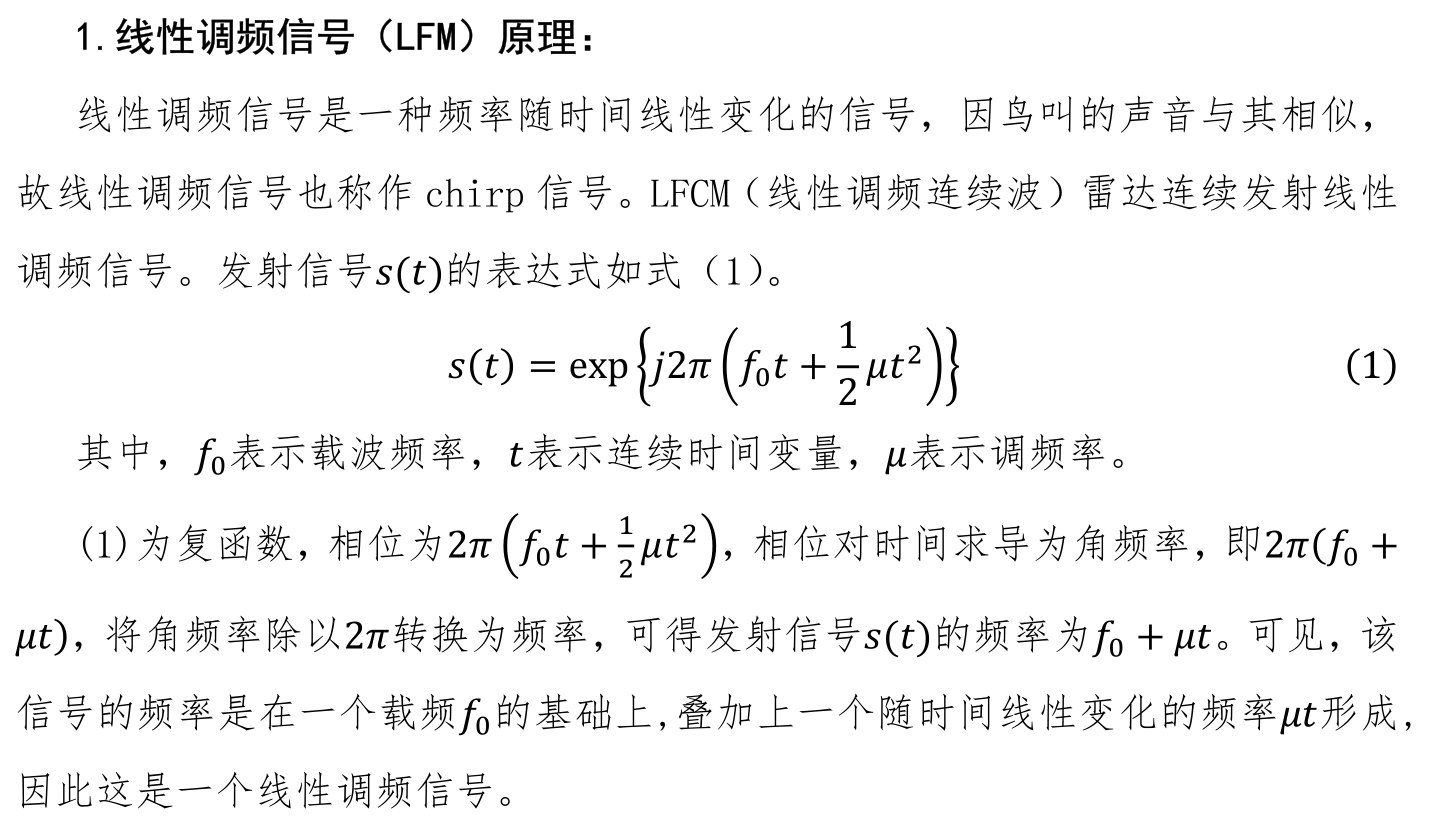2.线性调频信号（LFM）产生：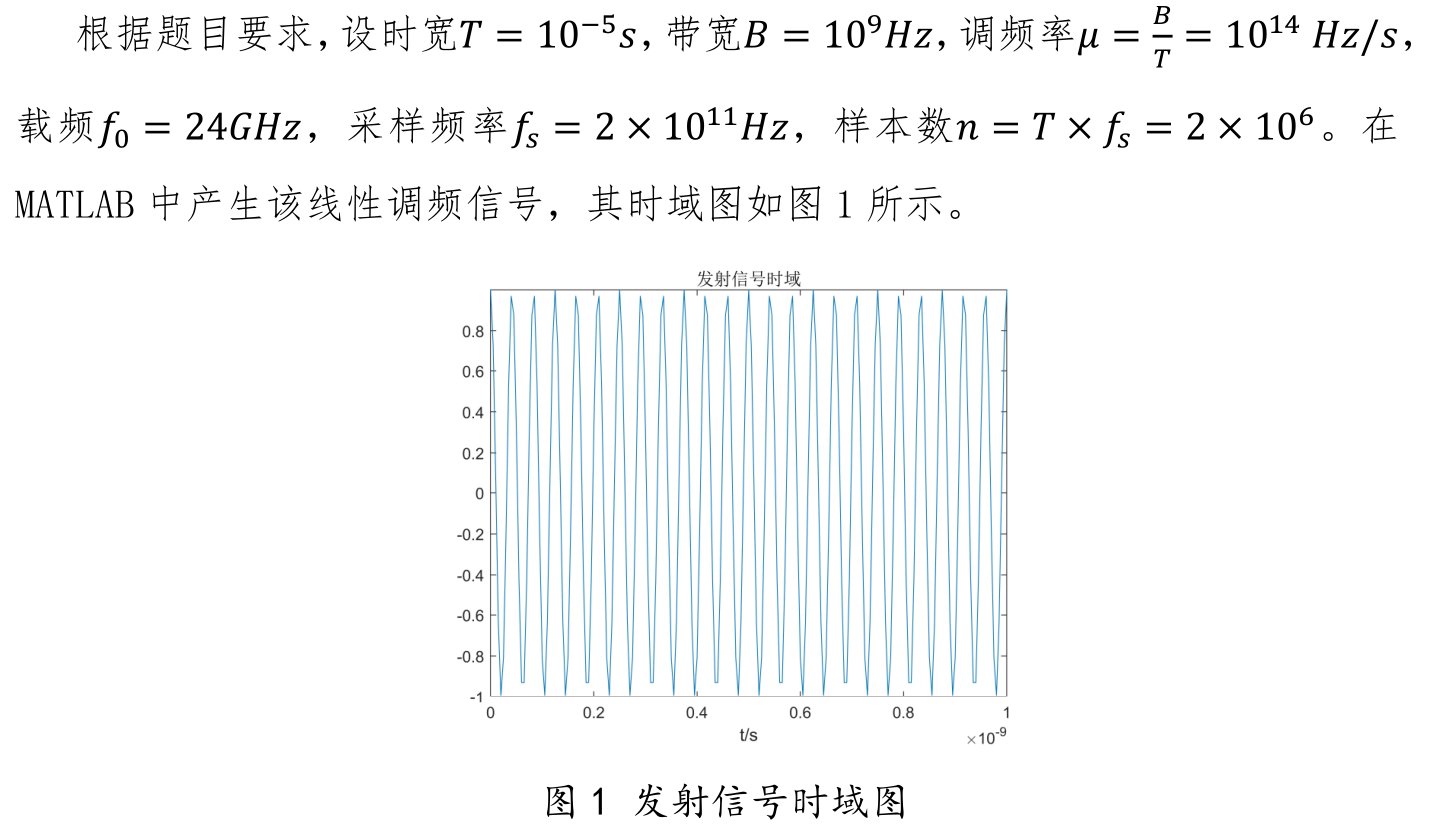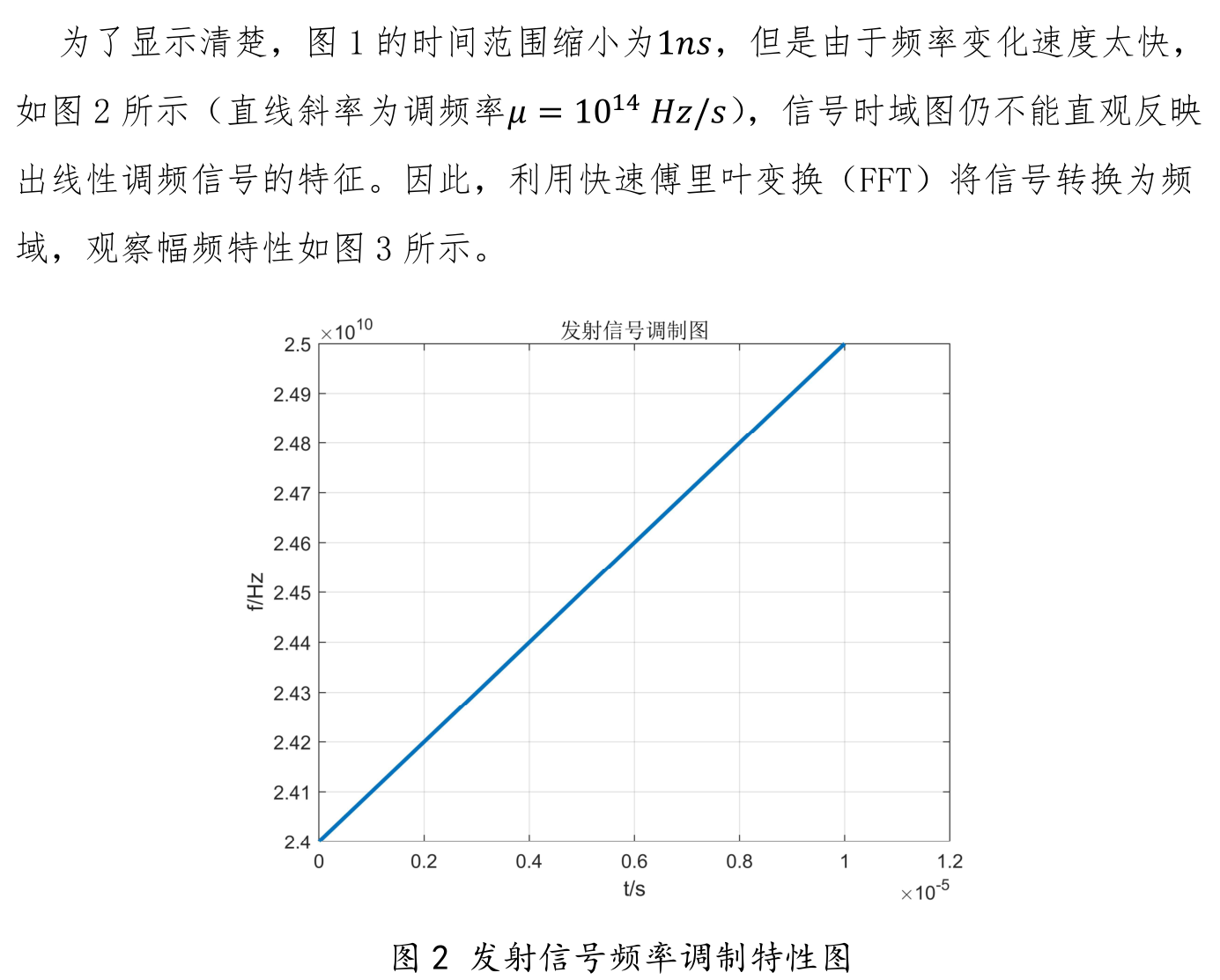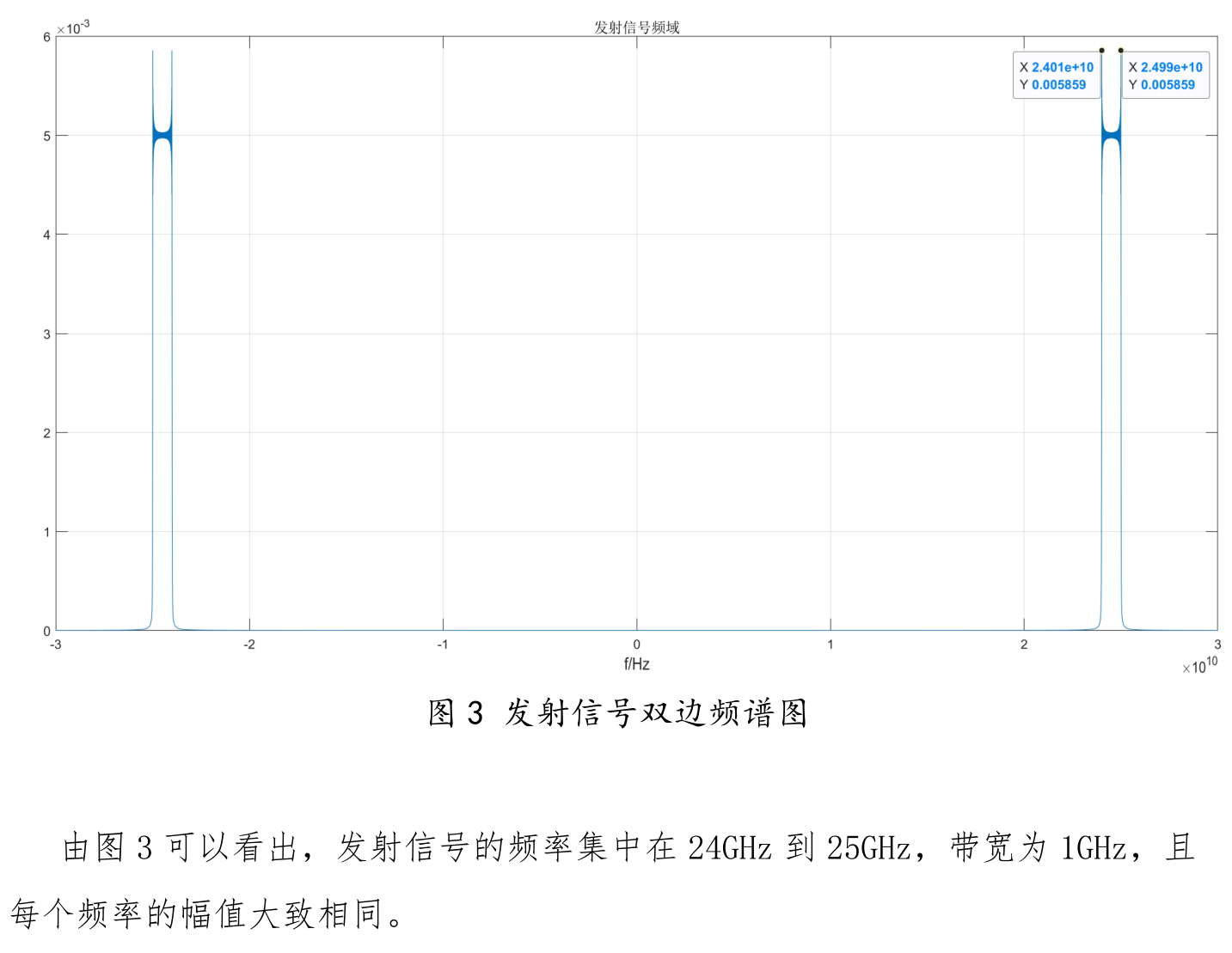3.雷达回波产生：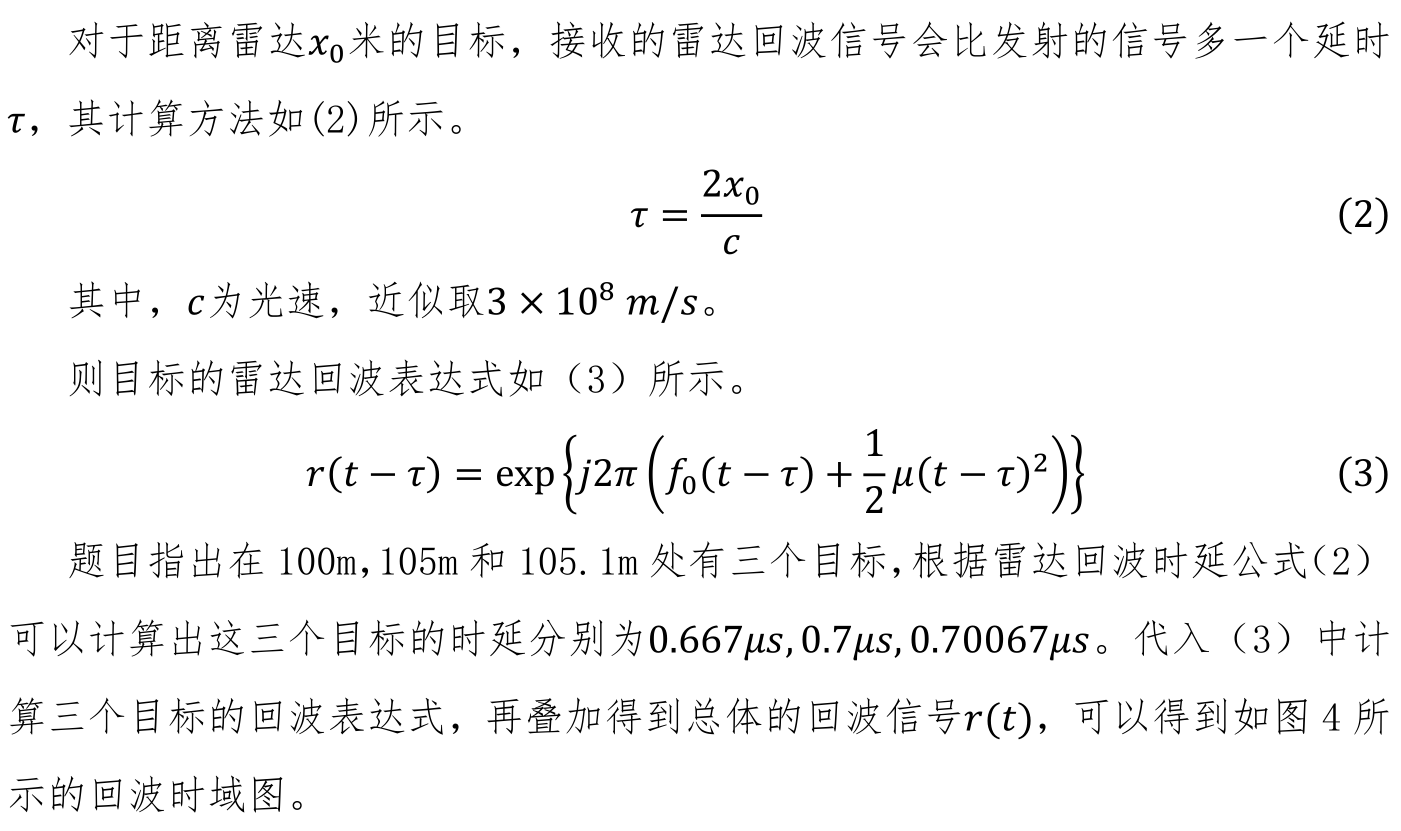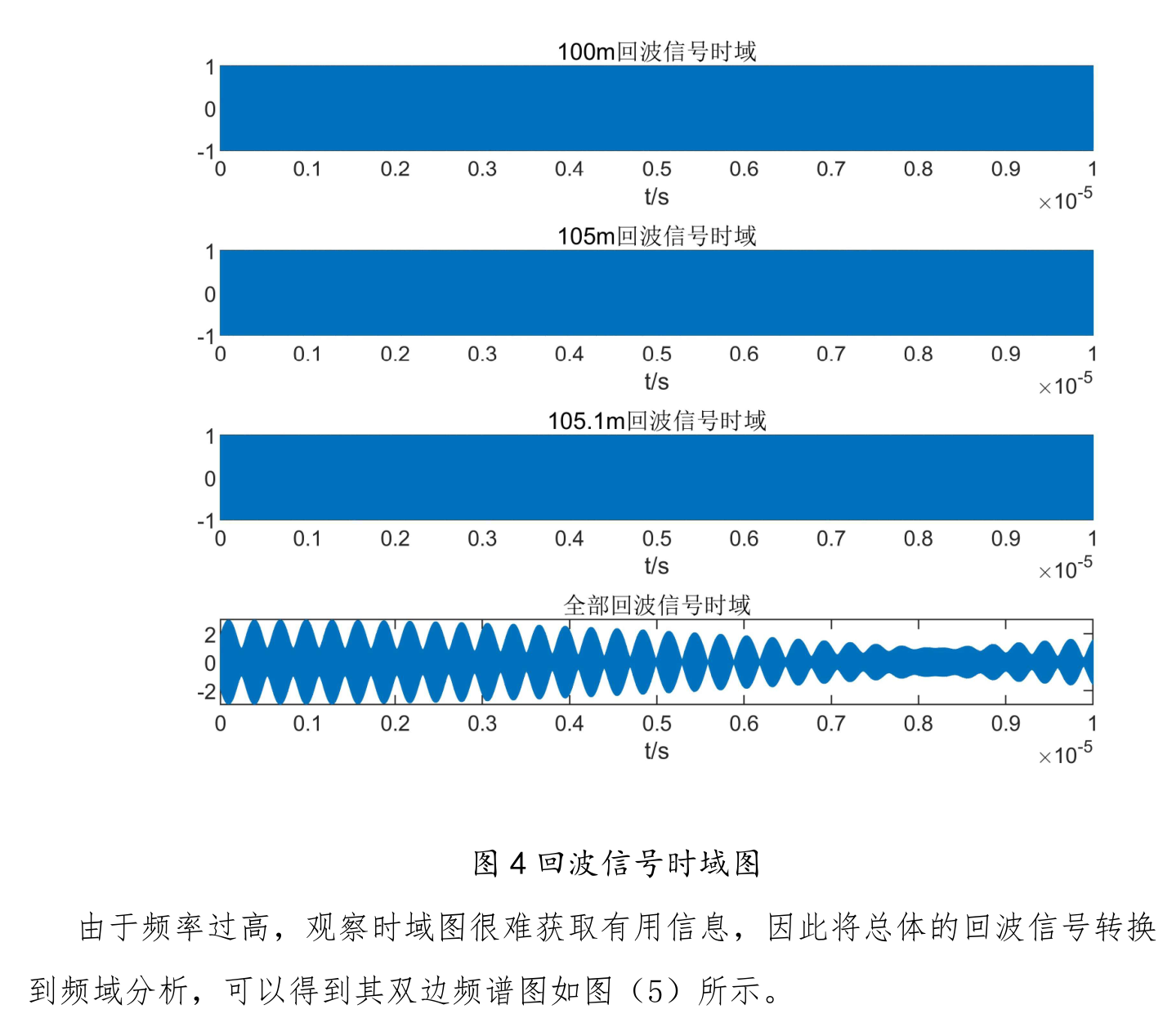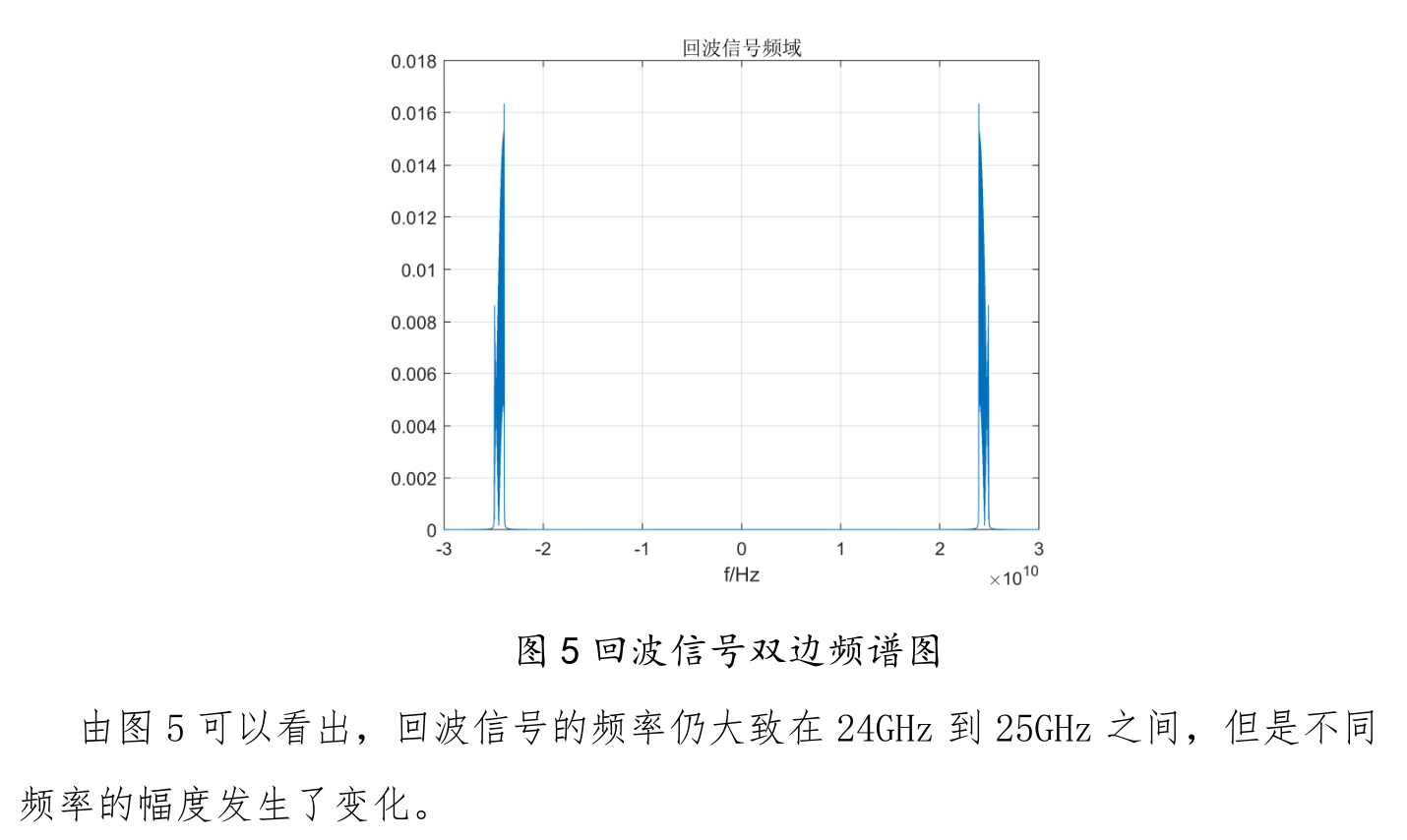4.下混频原理：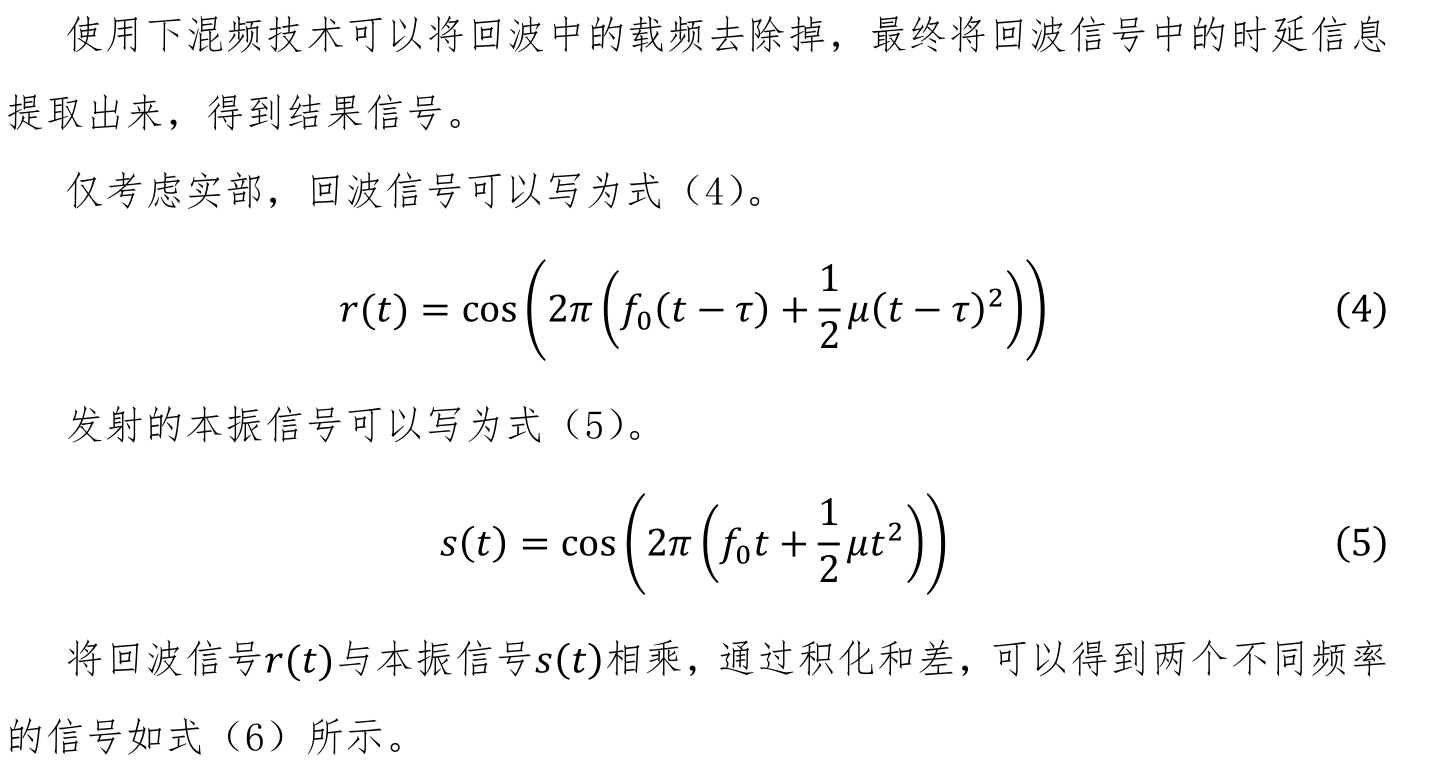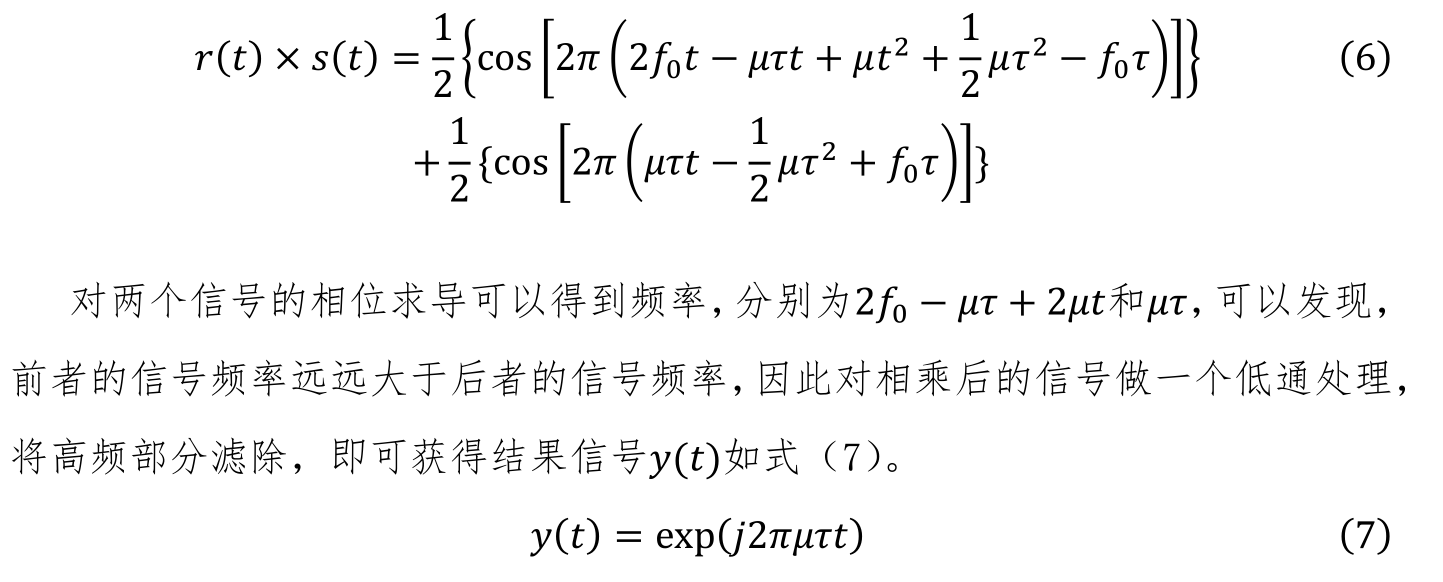5.下混频实现：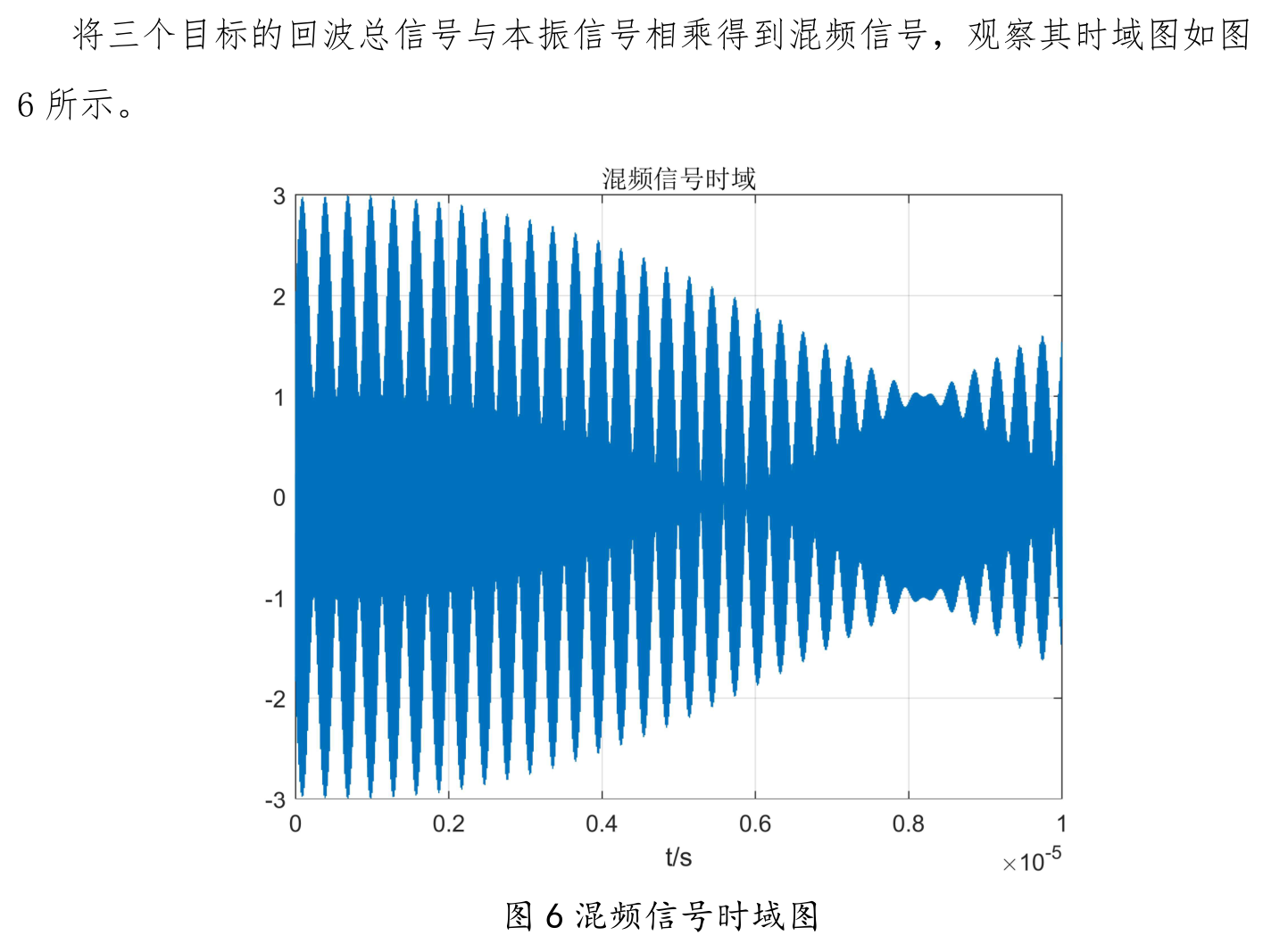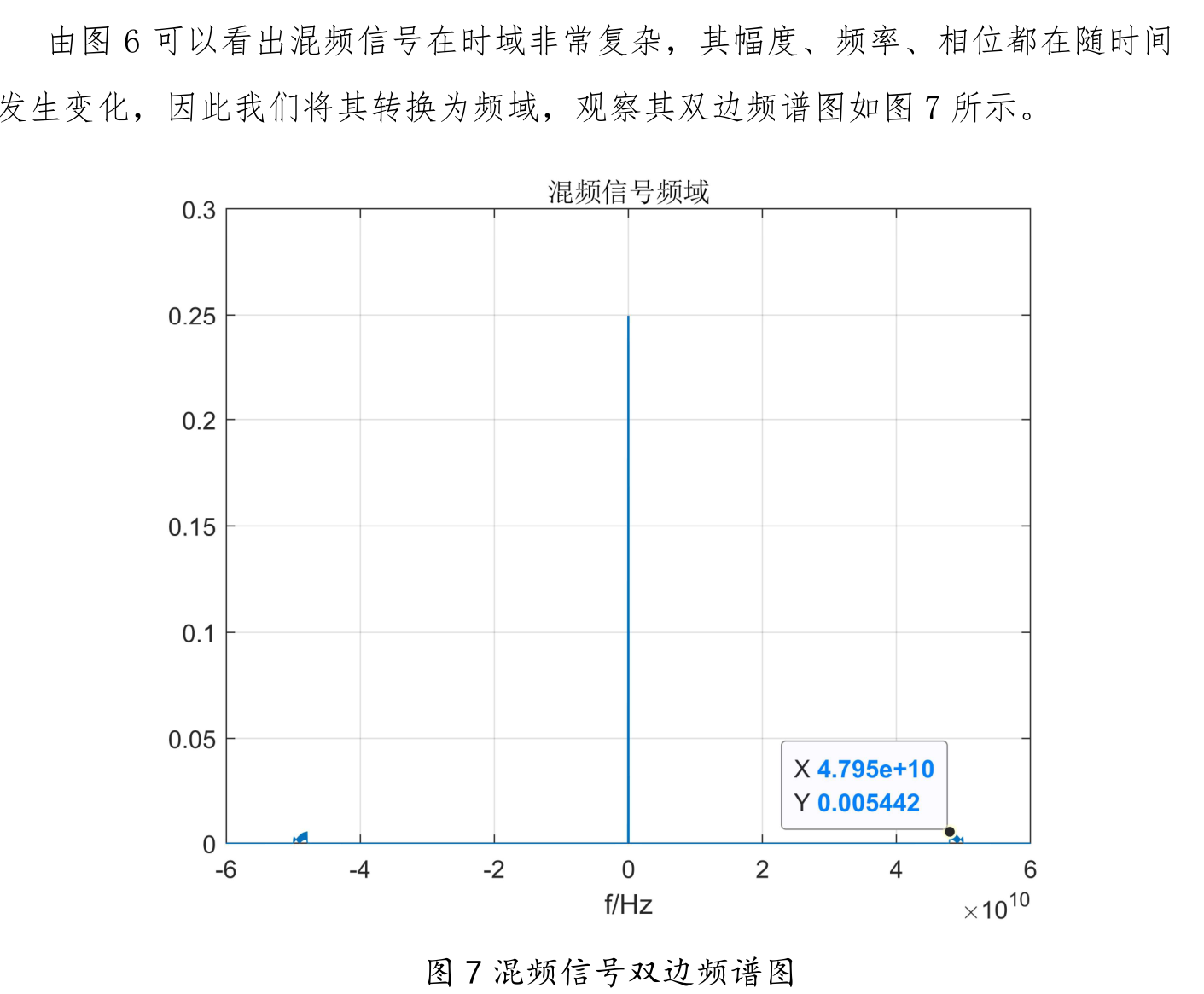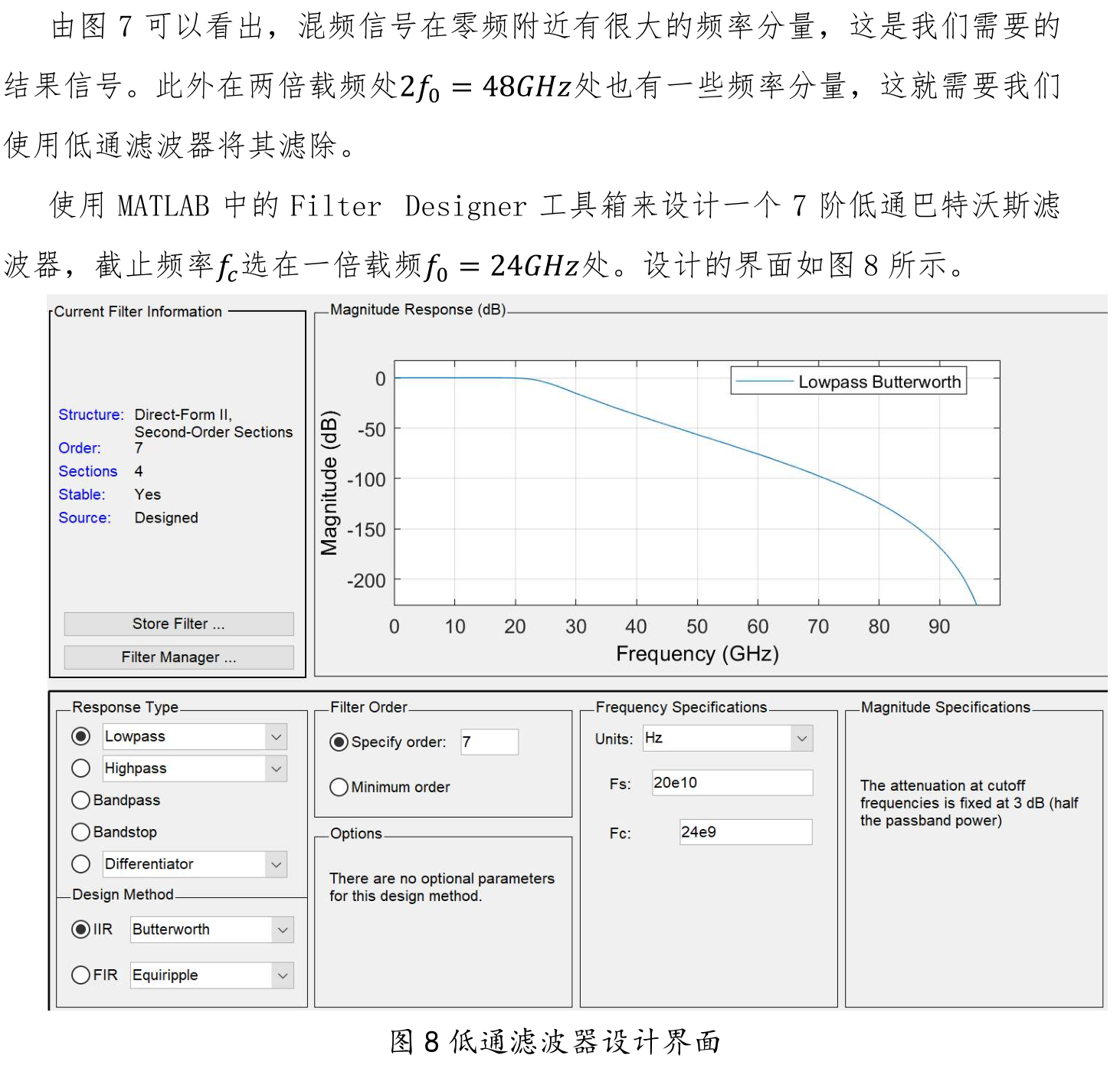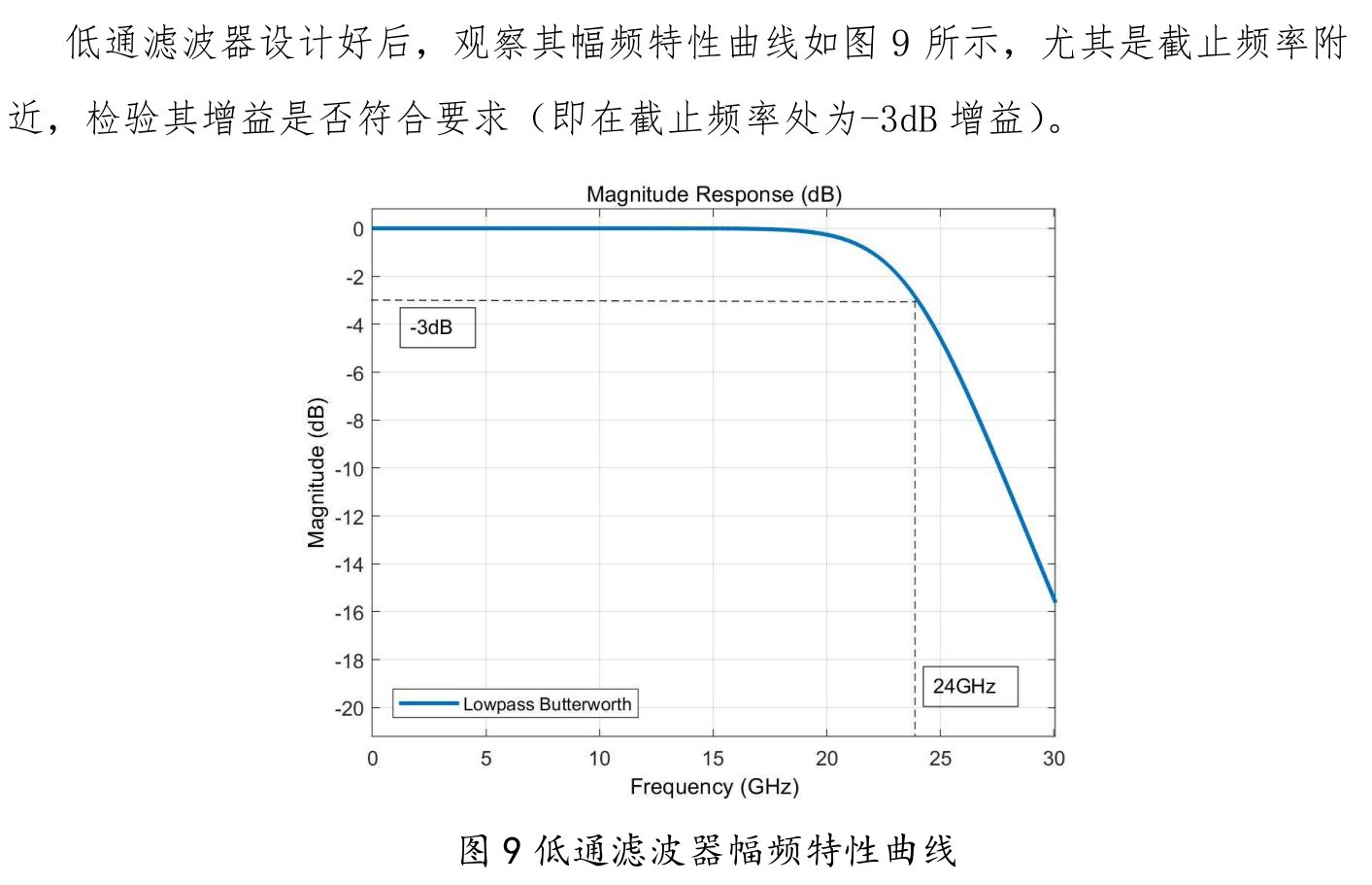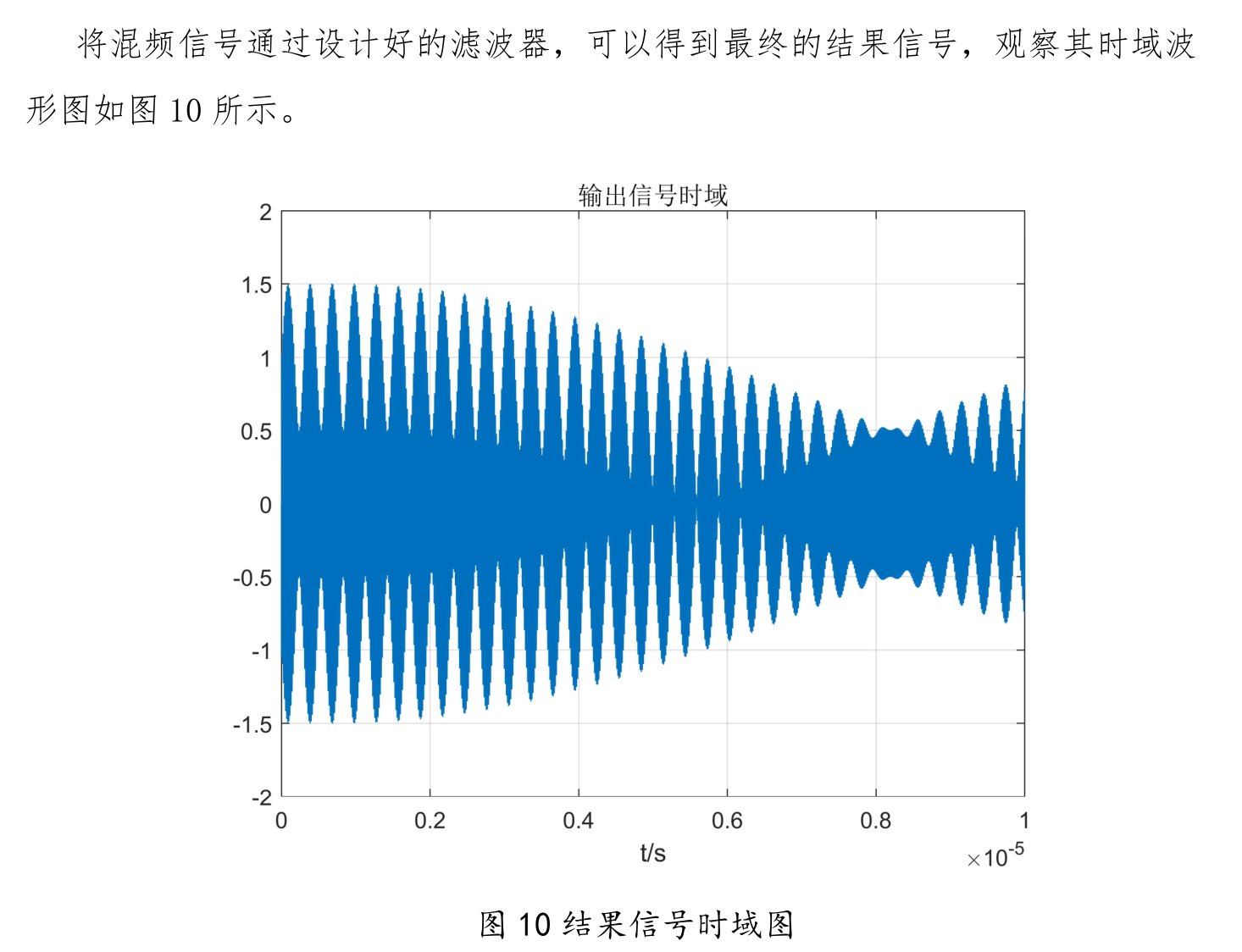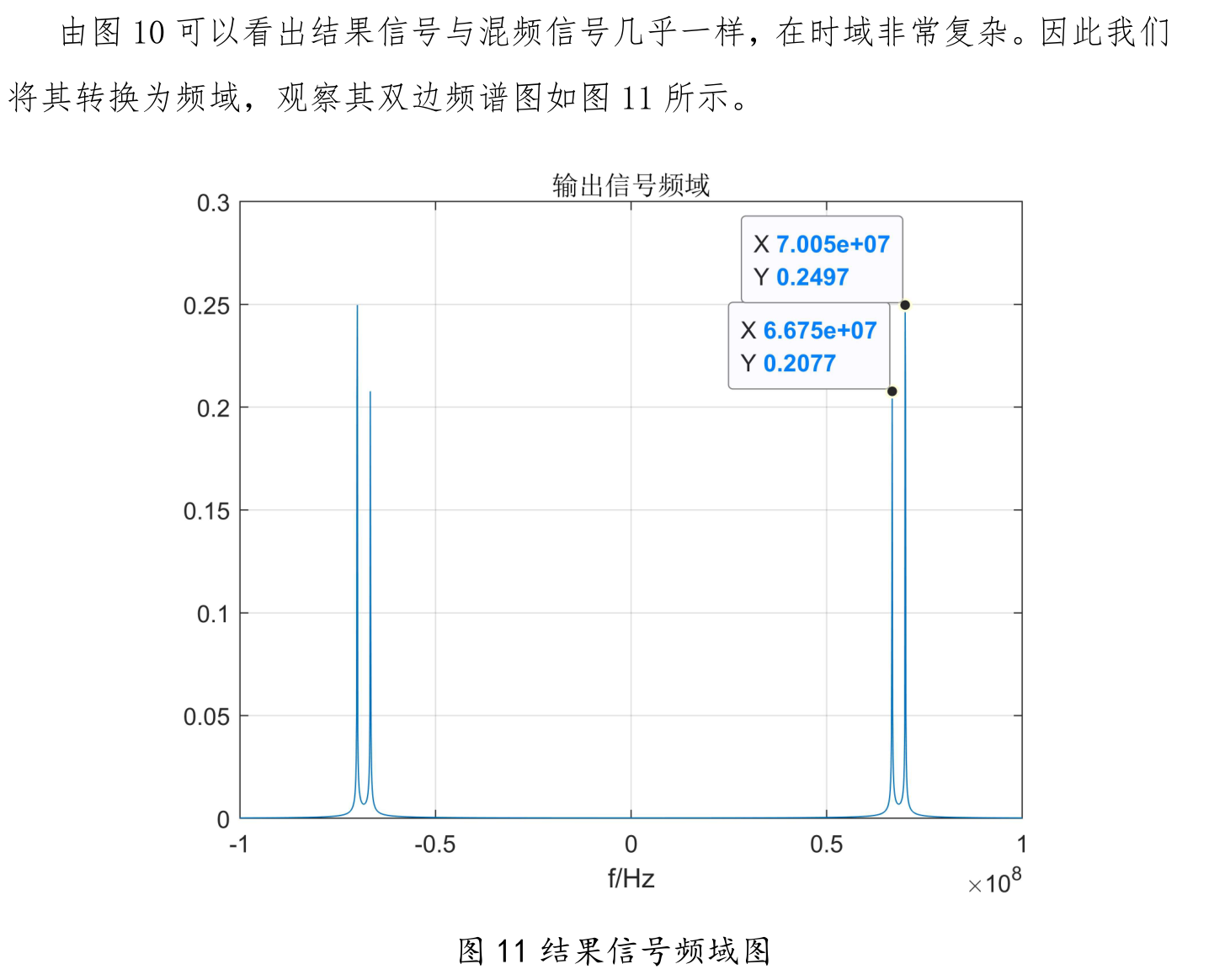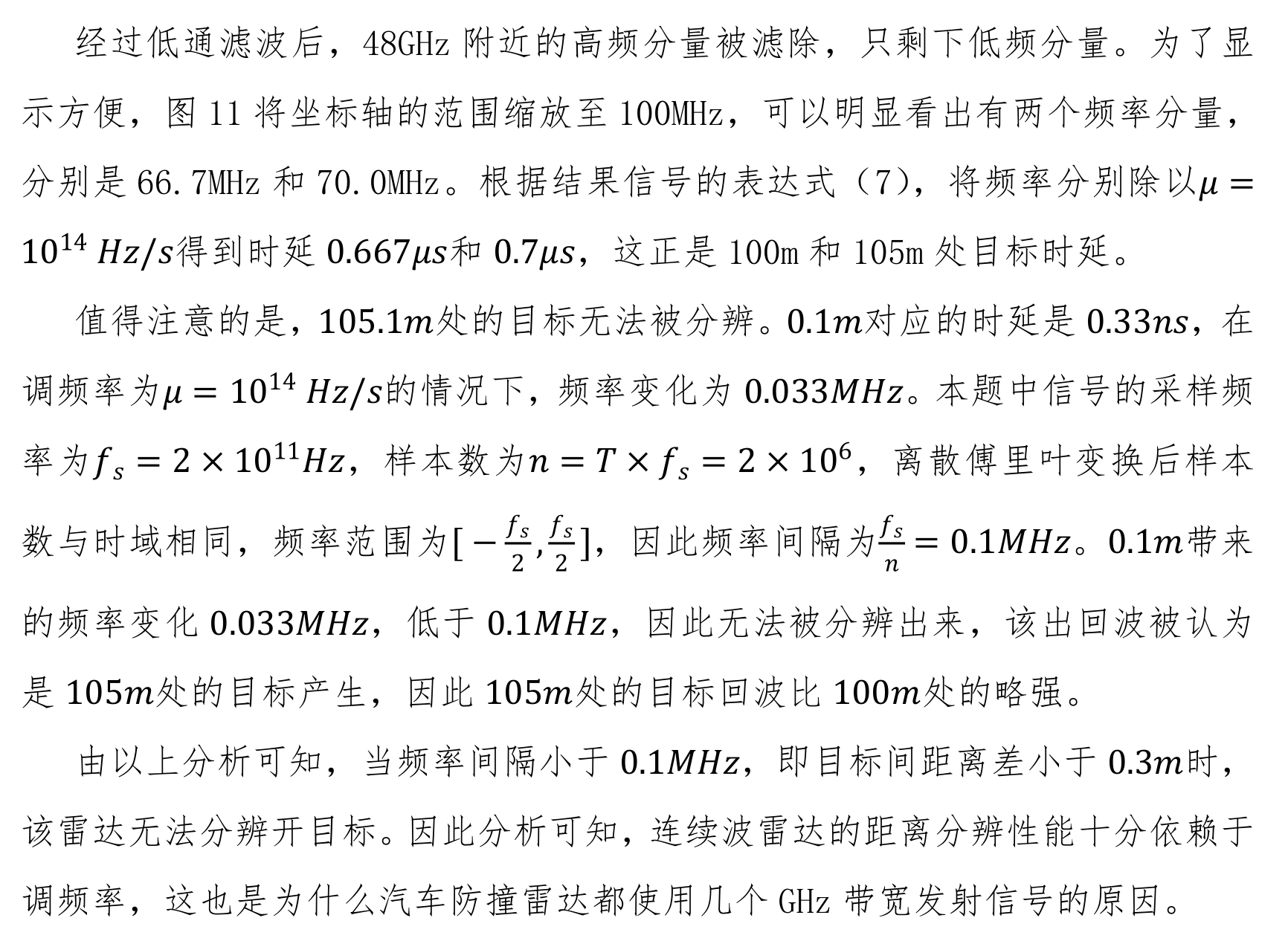三、心得感悟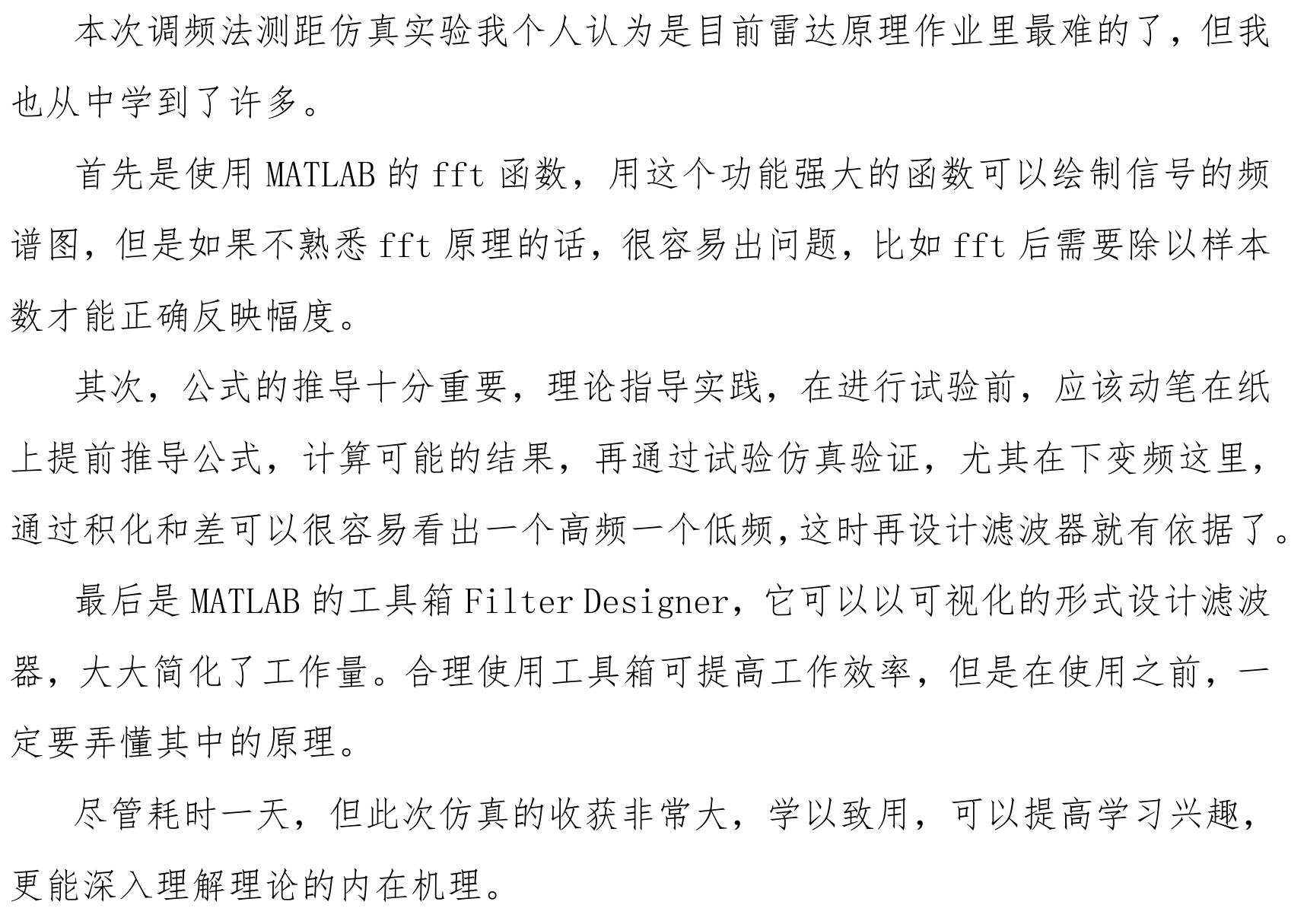虽说截图写博客确实不好，但是确实太困了。如果有需要word版的可以评论或者私信找我。这些地方我也学的不是很懂，要是有发现可以改进的地方，欢迎评论区指正！
附全部代码：
fs=20e10;
T=10e-6;%时宽
B=1e9;%带宽
mu=B/T;%调频率
n=round(T*fs);%采样点个数
t=linspace(0,T,n);
f0=24e9;%载频
s=exp(2j*pi*(f0*t+0.5*mu*t.^2));
plot(t,real(s))%时域图
title("发射信号时域")
xlabel("t/s")
xlim([0,1e-9])

figure(2)
S=fftshift(fft(real(s))./n);
f=linspace(-fs/2,fs/2-1,n);%频域横坐标，注意奈奎斯特采样定理，最大原信号最大频率不超过采样频率的一半
plot(f,abs(S))%频域图
title("发射信号频域")
xlim([-3e10,3e10])
xlabel("f/Hz")
grid on

figure(3)
w=f0+mu.*t;
plot(t,w)%线性调频图
grid on
title("发射信号调制图")
xlabel("t/s")
ylabel("f/Hz")

pos=[100,105,105.1];%三个目标的位置/m
timedelay=2*pos/(3e8);%雷达回波时延
r1=exp(2j*pi*(f0*(t-timedelay(1))+0.5*mu*(t-timedelay(1)).^2));
r2=exp(2j*pi*(f0*(t-timedelay(2))+0.5*mu*(t-timedelay(2)).^2));
r3=exp(2j*pi*(f0*(t-timedelay(3))+0.5*mu*(t-timedelay(3)).^2));
figure(4)
subplot(411)
plot(t,real(r1))%时域图
title("100m回波信号时域")
xlim([0,1e-5])
xlabel("t/s")
subplot(412)
plot(t,real(r2))%时域图
xlim([0,1e-5])
title("105m回波信号时域")
xlabel("t/s")
subplot(413)
plot(t,real(r3))%时域图
xlim([0,1e-5])
title("105.1m回波信号时域")
xlabel("t/s")
r=r1+r2+r3;%回波信号加在一起
subplot(414)
plot(t,real(r))%时域图
xlim([0,1e-5])
title("全部回波信号时域")
xlabel("t/s")

figure(5)
R=fftshift(fft(real(r))./n);
f=linspace(-fs/2,fs/2-1,n);%频域横坐标，注意奈奎斯特采样定理，最大原信号最大频率不超过采样频率的一半
plot(f,abs(R))%频域图
title("回波信号频域")
xlim([-3e10,3e10])
xlabel("f/Hz")
grid on

%回波信号乘以本振信号做下混频
mix=real(s).*real(r);
figure(6)
plot(t,real(mix))%时域图
title("混频信号时域")
xlabel("t/s")
xlim([0,1e-5])
grid on

figure(7)
MIX=fftshift(fft(mix)./n);
f=linspace(-fs/2,fs/2-1,n);%频域横坐标，注意奈奎斯特采样定理，最大原信号最大频率不超过采样频率的一半
plot(f,abs(MIX))%频域图
title("混频信号频域")
xlim([-6e10,6e10])
ylim([0,0.3])
xlabel("f/Hz")
grid on

y=filter(butterLow,mix);%用设计好的截止频率24GHz的低通滤波器滤波
figure(8)
plot(t,real(y))%时域图
title("输出信号时域")
xlabel("t/s")
xlim([0,1e-5])
grid on

figure(9)
Y=fftshift(fft(y)./n);
f=linspace(-fs/2,fs/2-1,n);%频域横坐标，注意奈奎斯特采样定理，最大原信号最大频率不超过采样频率的一半
plot(f,abs(Y))%频域图
title("输出信号频域")
xlim([-10^8,10^8])
ylim([0,0.3])
xlabel("f/Hz")
grid on


其中butterLow滤波器代码：
function Hd = butterLow
%BUTTERLOW Returns a discrete-time filter object.

% MATLAB Code
% Generated by MATLAB(R) 9.5 and Signal Processing Toolbox 8.1.
% Generated on: 21-Apr-2021 11:52:59

% Butterworth Lowpass filter designed using FDESIGN.LOWPASS.

% All frequency values are in Hz.
Fs = 2e+11;  % Sampling Frequency

N  = 7;            % Order
Fc = 24000000000;  % Cutoff Frequency

% Construct an FDESIGN object and call its BUTTER method.
h  = fdesign.lowpass('N,F3dB', N, Fc, Fs);
Hd = design(h, 'butter');

% [EOF]


留个赞再走啊，不然都没有信心写下一篇博客了\emoji{said}


展开全文信号处理 fft
• 线性调频 LFM脉冲压缩雷达仿真 一 雷达工作原理 雷达是 Radar RAdio Detection And Ranging 的音译词意为无线电检测和测距 即 利用无线电波来检测目标并测定目标的位置 这也是雷达设备在最初阶段的功能 典型的雷 达...
《线性调频(LFM)仿真》由会员分享，可在线阅读，更多相关《线性调频(LFM)仿真(14页珍藏版)》请在人人文库网上搜索。1、线性调频(LFM)脉冲压缩雷达仿真一 雷达工作原理雷达是Radar(RAdio Detection And Ranging)的音译词，意为“无线电检测和测距”，即利用无线电波来检测目标并测定目标的位置，这也是雷达设备在最初阶段的功能。典型的雷达系统如图1.1，它主要由发射机，天线，接收机，数据处理，定时控制，显示等设备组成。利用雷达可以获知目标的有无，目标斜距，目标角位置，目标相对速度等。现代高分辨雷达扩展了原始雷达概念，使它具有对运动目标(飞机，导弹等)和区域目标(地面等)成像和识别的能力。雷达的应用越来越广泛。图1.1：简单脉冲雷达系统框图雷达发射机的任务是产生符合要求的雷达波形(Rada。2、r Waveform)，然后经馈线和收发开关由发射天线辐射出去，遇到目标后，电磁波一部分反射，经接收天线和收发开关由接收机接收，对雷达回波信号做适当的处理就可以获知目标的相关信息。假设理想点目标与雷达的相对距离为R，为了探测这个目标，雷达发射信号,电磁波以光速向四周传播，经过时间后电磁波到达目标，照射到目标上的电磁波可写成：。电磁波与目标相互作用，一部分电磁波被目标散射，被反射的电磁波为，其中为目标的雷达散射截面(Radar Cross Section ,简称RCS)，反映目标对电磁波的散射能力。再经过时间后，被雷达接收天线接收的信号为。如果将雷达天线和目标看作一个系统，便得到如图1.2的等效。3、，而且这是一个LTI(线性时不变)系统。图1.2：雷达等效于LTI系统等效LTI系统的冲击响应可写成:(1.1)M表示目标的个数，是目标散射特性，是光速在雷达与目标之间往返一次的时间，(1.2)式中，为第i个目标与雷达的相对距离。雷达发射信号经过该LTI系统，得输出信号(即雷达的回波信号)：(1.3) 那么，怎样从雷达回波信号提取出表征目标特性的(表征相对距离)和(表征目标反射特性)呢？常用的方法是让通过雷达发射信号的匹配滤波器，如图1.3。 图1.3：雷达回波信号处理 的匹配滤波器为：(1.4)于是， (1.5)对上式进行傅立叶变换：(1.6)如果选取合适的，使它的幅频特性为常数，那么1.6。4、式可写为：(1.7)其傅立叶反变换为： (1.8)中包含目标的特征信息和。从 中可以得到目标的个数M和每个目标相对雷达的距离： (1.9)这也是线性调频(LFM)脉冲压缩雷达的工作原理。二 线性调频(LFM)信号脉冲压缩雷达能同时提高雷达的作用距离和距离分辨率。这种体制采用宽脉冲发射以提高发射的平均功率，保证足够大的作用距离；而接受时采用相应的脉冲压缩算法获得窄脉冲，以提高距离分辨率，较好的解决雷达作用距离与距离分辨率之间的矛盾。脉冲压缩雷达最常见的调制信号是线性调频(Linear Frequency Modulation)信号,接收时采用匹配滤波器(Matched Filter)压缩脉冲。L。5、FM信号(也称Chirp 信号)的数学表达式为：(2.1)式中为载波频率，为矩形信号，(2.2) ，是调频斜率，于是，信号的瞬时频率为，如图2.1 图2.1 典型的chirp信号(a)up-chirp(K0)(b)down-chirp(K0)将2.1式中的up-chirp信号重写为：(2.3)式中，(2.4)是信号s(t)的复包络。由傅立叶变换性质，S(t)与s(t)具有相同的幅频特性，只是中心频率不同而以，因此，Matlab仿真时，只需考虑S(t)。以下Matlab程序产生2.4式的chirp信号，并作出其时域波形和幅频特性，如图2.2。%demo of chirp signalT=10e-。6、6; %pulse duration10usB=30e6; %chirp frequency modulation bandwidth 30MHzK=B/T; %chirp slopeFs=2*B;Ts=1/Fs; %sampling frequency and sample spacingN=T/Ts;t=linspace(-T/2,T/2,N);St=exp(j*pi*K*t.2); %generate chirp signalsubplot(211)plot(t*1e6,real(St);xlabel(Time in u sec);title(Real part of chirp sig。7、nal);grid on;axis tight;subplot(212)freq=linspace(-Fs/2,Fs/2,N);plot(freq*1e-6,fftshift(abs(fft(St);xlabel(Frequency in MHz);title(Magnitude spectrum of chirp signal);grid on;axis tight;仿真结果显示：图2.2：LFM信号的时域波形和幅频特性三 LFM脉冲的匹配滤波信号的匹配滤波器的时域脉冲响应为：(3.1)是使滤波器物理可实现所附加的时延。理论分析时，可令0，重写3.1式，(3.2)将2.1式代入3.2式得:(。8、3.3 )图3.1：LFM信号的匹配滤波如图3.1,经过系统得输出信号，当时,(3.4)当时,(3.5)合并3.4和3.5两式：(3.6)3.6式即为LFM脉冲信号经匹配滤波器得输出,它是一固定载频的信号。当时，包络近似为辛克(sinc)函数。(3.7)图3.2：匹配滤波的输出信号如图3.2，当时，为其第一零点坐标；当时，习惯上，将此时的脉冲宽度定义为压缩脉冲宽度。(3.8)LFM信号的压缩前脉冲宽度T和压缩后的脉冲宽度之比通常称为压缩比D，(3.9)3.9式表明，压缩比也就是LFM信号的时宽频宽积。由2.1,3.3,3.6式，s(t),h(t),so(t)均为复信号形式，Matab仿真时，只。9、需考虑它们的复包络S(t),H(t),So(t)。以下Matlab程序段仿真了图3.1所示的过程，并将仿真结果和理论进行对照。%demo of chirp signal after matched filterT=10e-6; %pulse duration10usB=30e6; %chirp frequency modulation bandwidth 30MHzK=B/T; %chirp slopeFs=10*B;Ts=1/Fs; %sampling frequency and sample spacingN=T/Ts;t=linspace(-T/2,T/2,N);St=exp(j*pi*。10、K*t.2); %chirp signalHt=exp(-j*pi*K*t.2); %matched filterSot=conv(St,Ht); %chirp signal after matched filtersubplot(211)L=2*N-1;t1=linspace(-T,T,L);Z=abs(Sot);Z=Z/max(Z); %normalize Z=20*log10(Z+1e-6);Z1=abs(sinc(B.*t1); %sinc functionZ1=20*log10(Z1+1e-6);t1=t1*B; plot(t1,Z,t1,Z1,r.);axis(-15,15,-50。11、,inf);grid on;legend(emulational,sinc);xlabel(Time in sec timesitB);ylabel(Amplitude,dB);title(Chirp signal after matched filter);subplot(212) %zoomN0=3*Fs/B;t2=-N0*Ts:Ts:N0*Ts;t2=B*t2;plot(t2,Z(N-N0:N+N0),t2,Z1(N-N0:N+N0),r.);axis(-inf,inf,-50,inf);grid on;set(gca,Ytick,-13.4,-4,0,Xtick,-3,-2,-1,-0。12、.5,0,0.5,1,2,3);xlabel(Time in sec timesitB);ylabel(Amplitude,dB);title(Chirp signal after matched filter (Zoom);仿真结果如图3.3：图3.3：Chirp信号的匹配滤波图3.3中，时间轴进行了归一化，()。图中反映出理论与仿真结果吻合良好。第一零点出现在(即)处，此时相对幅度-13.4dB。压缩后的脉冲宽度近似为()，此时相对幅度-4dB,这理论分析(图3.2)一致。上面只是对各个信号复包络的仿真，实际雷达系统中，LFM脉冲的处理过程如图3.4。图3.4： LFM信号的接收处理过程雷。13、达回波信号(1.4式)经过正交解调后，得到基带信号，再经过匹配滤波脉冲压缩后就可以作出判决。正交解调原理如图3.5，雷达回波信号经正交解调后得两路相互正交的信号I(t)和Q(t)。一种数字方法处理的的匹配滤波原理如图3.6。图3.5：正交解调原理图3.6：一种脉冲压缩雷达的数字处理方式四：Matlab仿真结果(1)任务：对以下雷达系统仿真。雷达发射信号参数：幅度：1.0信号波形：线性调频信号频带宽度：30兆赫兹(30MHz)脉冲宽度：10微妙(20us)中心频率：1GHz(109Hz)雷达接收方式：正交解调接收距离门：10Km15Km目标：Tar1：10.5KmTar2：11KmTar3：12。14、KmTar4：12Km5mTar5：13KmTar6：13Km2m(2)系统模型：结合以上分析，用Matlab仿真雷达发射信号，回波信号，和压缩后的信号的复包络特性，其载频不予考虑(实际中需加调制和正交解调环节)，仿真信号与系统模型如图4.1。图4.1：雷达仿真等效信号与系统模型(3)线性调频脉冲压缩雷达仿真程序LFM_radar仿真程序模拟产生理想点目标的回波，并采用频域相关方法(以便利用FFT)实现脉冲压缩。函数LFM_radar的参数意义如下：T：chirp信号的持续脉宽；B：chirp信号的调频带宽；Rmin：观测目标距雷达的最近位置；Rmax：观测目标距雷达的最远位置；R：一维数组，。15、数组值表示每个目标相对雷达的斜距；RCS：一维数组，数组值表示每个目标的雷达散射截面。在Matlab指令窗中键入：LFM_radar(10e-6,30e6,10000,15000,10500,11000,12000,12005,13000,13002,1,1,1,1,1,1)得到的仿真结果如图4.2。(4)分辨率(Resolution)仿真改变两目标的相对位置，可以分析线性调频脉冲压缩雷达的分辨率。仿真程序默认参数的距离分辨率为：(4.1)图4.3为分辨率仿真结果，可做如下解释：(a) 图为单点目标压缩候的波形；(b) 图中，两目标相距2m，小于，因而不能分辨；(c) 图中，两目标相距5m，等。16、于，实际上是两目标的输出sinc包络叠加，可以看到他们的副瓣相互抵消；(d) (h)图中，两目标距离大于雷达的距离分辨率，可以观察出，它们的主瓣变宽，直至能分辨出两目标。图4.2：仿真结果图4.3：线性调频脉冲压缩雷达分辨率仿真附录：LFM_radar.m%demo of LFM pulse radar%=function LFM_radar(T,B,Rmin,Rmax,R,RCS)if nargin=0T=10e-6; %pulse duration 10usB=30e6; %chirp frequency modulation bandwidth 30MHzRmin=10000;Rmax=。17、15000; %range binR=10500,11000,12000,12008,13000,13002; %position of ideal point targetsRCS=1 1 1 1 1 1; %radar cross sectionend%=%ParameterC=3e8; %propagation speedK=B/T; %chirp slopeRwid=Rmax-Rmin; %receive window in meterTwid=2*Rwid/C; %receive window in secondFs=5*B;Ts=1/Fs; %sampling frequency 。18、and sampling spacingNwid=ceil(Twid/Ts); %receive window in number%=%Gnerate the echo t=linspace(2*Rmin/C,2*Rmax/C,Nwid); %receive window%open window when t=2*Rmin/C%close window when t=2*Rmax/C M=length(R); %number of targets td=ones(M,1)*t-2*R/C*ones(1,Nwid);Srt=RCS*(exp(j*pi*K*td.2).*(abs(td)T/2);。19、%radar echo from point targets %=%Digtal processing of pulse compression radar using FFT and IFFTNchirp=ceil(T/Ts); %pulse duration in numberNfft=2nextpow2(Nwid+Nwid-1)； %number needed to compute linear %convolution using FFT algorithmSrw=fft(Srt,Nfft); %fft of radar echot0=linspace(-T/2,T/2,Nchirp)。20、; St=exp(j*pi*K*t0.2); %chirp signal Sw=fft(St,Nfft); %fft of chirp signalSot=fftshift(ifft(Srw.*conj(Sw); %signal after pulse compression%=N0=Nfft/2-Nchirp/2;Z=abs(Sot(N0:N0+Nwid-1);Z=Z/max(Z);Z=20*log10(Z+1e-6);%figuresubplot(211)plot(t*1e6,real(Srt);axis tight;xlabel(Time in u sec);ylabel(Amplitude)title(Radar echo without compression);subplot(212)plot(t*C/2,Z)axis(10000,15000,-60,0);xlabel(Range in meters);ylabel(Amplitude in dB)title(Radar echo after compression);。
展开全文一、简介
1．雷达工作原理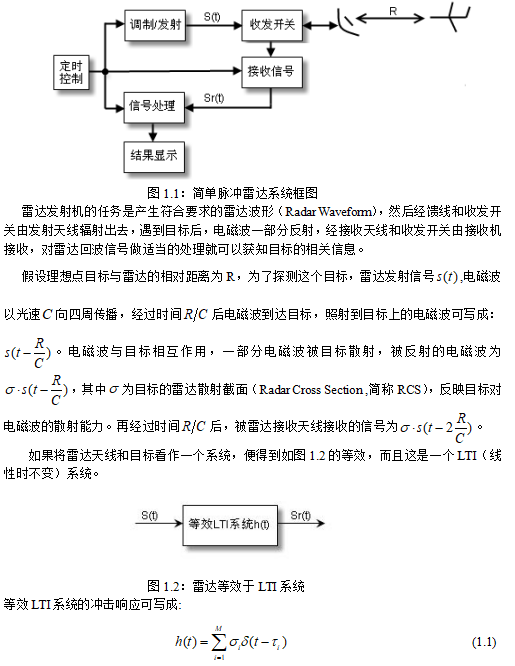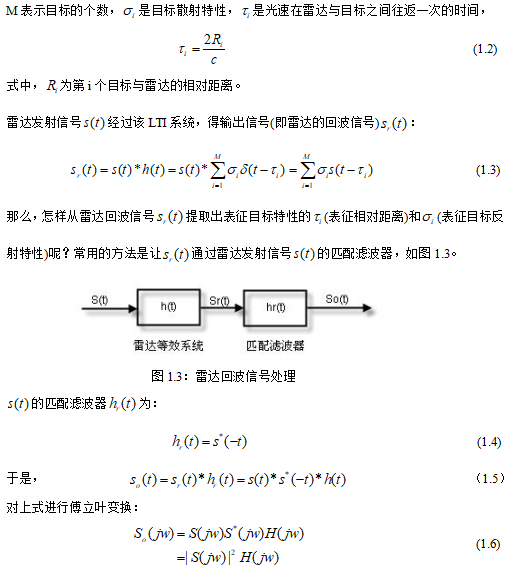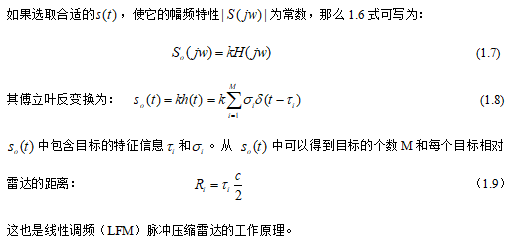2．线性调频（LFM）信号
脉冲压缩雷达能同时提高雷达的作用距离和距离分辨率。这种体制采用宽脉冲发射以提高发射的平均功率，保证足够大的作用距离；而接受时采用相应的脉冲压缩算法获得窄脉冲，以提高距离分辨率，较好的解决雷达作用距离与距离分辨率之间的矛盾。
脉冲压缩雷达最常见的调制信号是线性调频（Linear Frequency Modulation）信号,接收时采用匹配滤波器（Matched Filter）压缩脉冲。
LFM信号(也称Chirp 信号)的数学表达式为：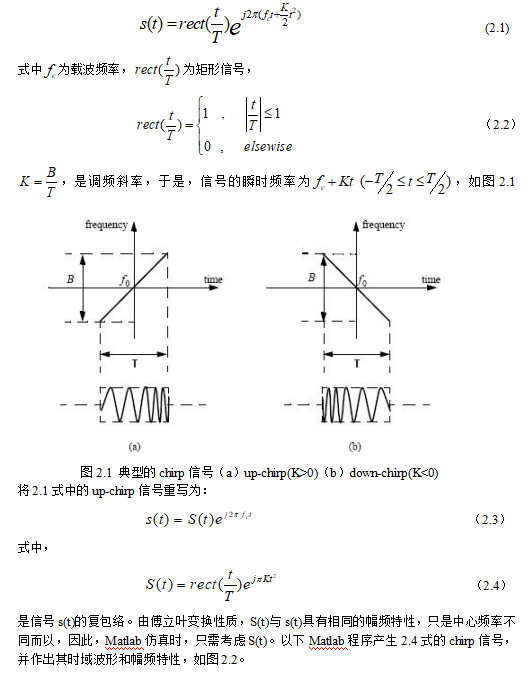3. LFM脉冲的匹配滤波
信号的匹配滤波器的时域脉冲响应为：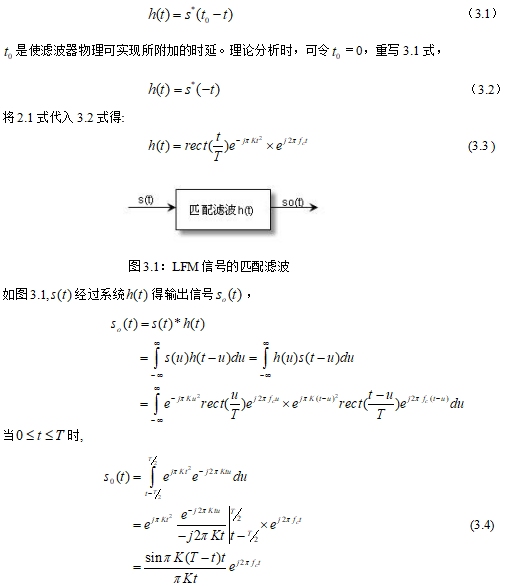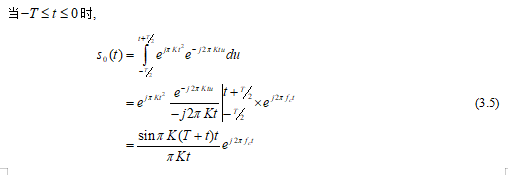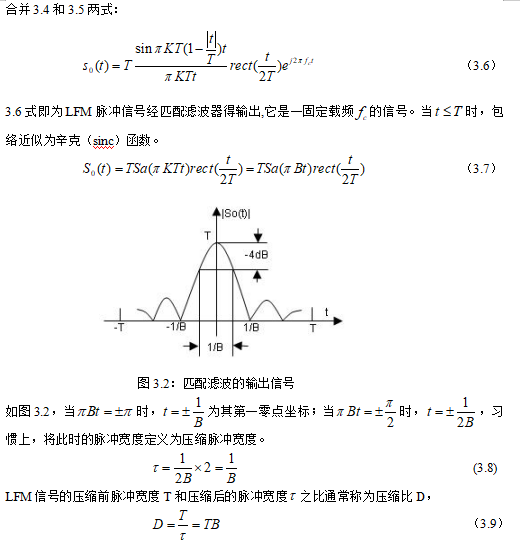二、源代码
%%demo of LFM pulse radar
%==================================================================
if nargin==0
T=10e-6;                                          %pulse duration 10us
B=30e6;                                           %chirp frequency modulation bandwidth 30MHz
Rmin=10000;Rmax=15000;              %range bin
R=[10500,11000,12000,12008,13000,13002];  %position of ideal point targets
RCS=[1 1 1 1 1 1];                           %radar cross section
end
%==================================================================
%%Parameter
C=3e8;                                            %propagation speed
K=B/T;                                             %chirp slope
Fs=5*B;Ts=1/Fs;                             %sampling frequency and sampling spacing
%==================================================================
%%Gnerate the echo
%open window when t=2*Rmin/C
%close window when t=2*Rmax/C
M=length(R);                                        %number of targets
td=ones(M,1)*t-2*R'/C*ones(1,Nwid);
%%demo of chirp signal
T=10e-6;                                  %pulse duration10us
B=30e6;                                   %chirp frequency modulation bandwidth 30MHz
K=B/T;                                      %chirp slope
Fs=2*B;Ts=1/Fs;                      %sampling frequency and sampling spacing
N=T/Ts;
t=linspace(-T/2,T/2,N);
St=exp(j*pi*K*t.^2);                    %generate chirp signal

三、运行结果四、备注
完整代码或者代写添加QQ912100926。
往期回顾>>>>>>
【信号处理】基于GUI界面之处理录音与音频【Matlab 123期】
【信号处理】CDR噪声和混响抑制【含Matlab源码 198期】
【信号处理】最小二乘法解决稀疏信号恢复问题【Matlab 199期】
【信号处理】遗传算法的VST混响【Matlab 200期】
【信号处理】HMM的睡眠状态检测【Matlab 201期】
【信号处理】小波变换的音频水印嵌入提取【Matlab 202期】
【信号处理】ICA算法信号分离【Matlab 203期】
【信号处理】基于GUI界面的脉搏信号之脉率存档【Matlab 204期】
【信号处理】基于GUI界面的虚拟信号发生器（各种波形）【Matlab 205期】
【信号处理】基于GUI界面信号发生器之电子琴【Matlab 206期】
【信号处理】数字电子琴设计与实现【Matlab 207期】
【雷达通信】雷达数字信号处理【Matlab 214期】


展开全文• 基于MATLAB平台以线性调频信号为例通过仿真研究了雷达信号处理中的脉冲压缩技术。在对线性调频信号时域波形进行仿真的基础上介绍了数字正交相干检波技术。最后基于匹配滤波算法对雷达回波信号进行了脉冲压缩仿真，...
• 在 FPGA 实现线性三角波调频信号产生、 数字下变频和 FFT 处理。 另外还对系 统所使用的 EMIF 接口实现进行阐述。 最后对系统验证测试及结果进行分析， 测 试结果符合预期要求本文通过对调频连续波雷达测距理论的...
• MATLAB程序利用线性调频信号的脉冲压缩技术实现对目标的高分辨率一维测距
• MTDDSP实验课大作业设计一 实验目的在DSP上实现线性调频信号的脉冲压缩、动目标显示(MTI)和动目标检测(MTD)，并将结果与MATLAB上的结果进行误差仿真。二 实验内容2.1 MATLAB仿真设定带宽、脉宽、采样率、脉冲重复...
• 0067、同步电机模型的MATLAB仿真论文资料 0068、危险气体泄露报警器设计论文资料 0069、微型打印机控制电路的设计论文资料 0070、温度监控系统的设计论文资料 0071、温度控制系统设计论文资料 0072、无线调频发射器...# 线性调频测距matlabmatlab 订阅